# PROBLEM 1 - Rescorla-Wagner Model

Link of the iPython notebook for the code

Let us model a classical conditioning experiment with resort to the Rescorla-Wagner rule.

The experiment is as follows: at trial $i ∈ ℕ$:

• an animal may or may not be presented with a stimulus $u_i$ (e.g.: ringing a bell, clapping hands): $u_i = 1$ if the stimulus is present, $u_i = 0$ otherwise
• … which may or may not in turn be followed by a reward $r_i$ (e.g.: food): $r_i = 1$ if there is a reward, $r_i = 0$ otherwise

The animal is assumed to be willing to predict the correlation between the stimulus and the reward (e.g: by salivating in anticipation): i.e. to predict if it will get the reward based on the presence or absence of the stimulus. As such, the prediction of the animal is denoted by $v_i$ at trial $i$:

$∀i, \; v_i ≝ w_i u_i$

where $w$ is a parameter learned and updated - trial after trial - by the animal, in accordance with the following Rescola-Wagner rule:

$w_i → w_i + \underbrace{ε}_{\text{learning rate } << 1} \underbrace{(r_i − v_i)}_{≝ \, δ_i} u_i$

## 1. Introductory example

For instance, let us suppose that there are $50$ trials, and:

• during the first $25$ trials, both stimuli and reward are present ($u_i = r_i = 1$)
• during the last $25$ ones, only the stimulus is present ($u_i = 1, \, r_i = 0$)

as illustrated by the following figure: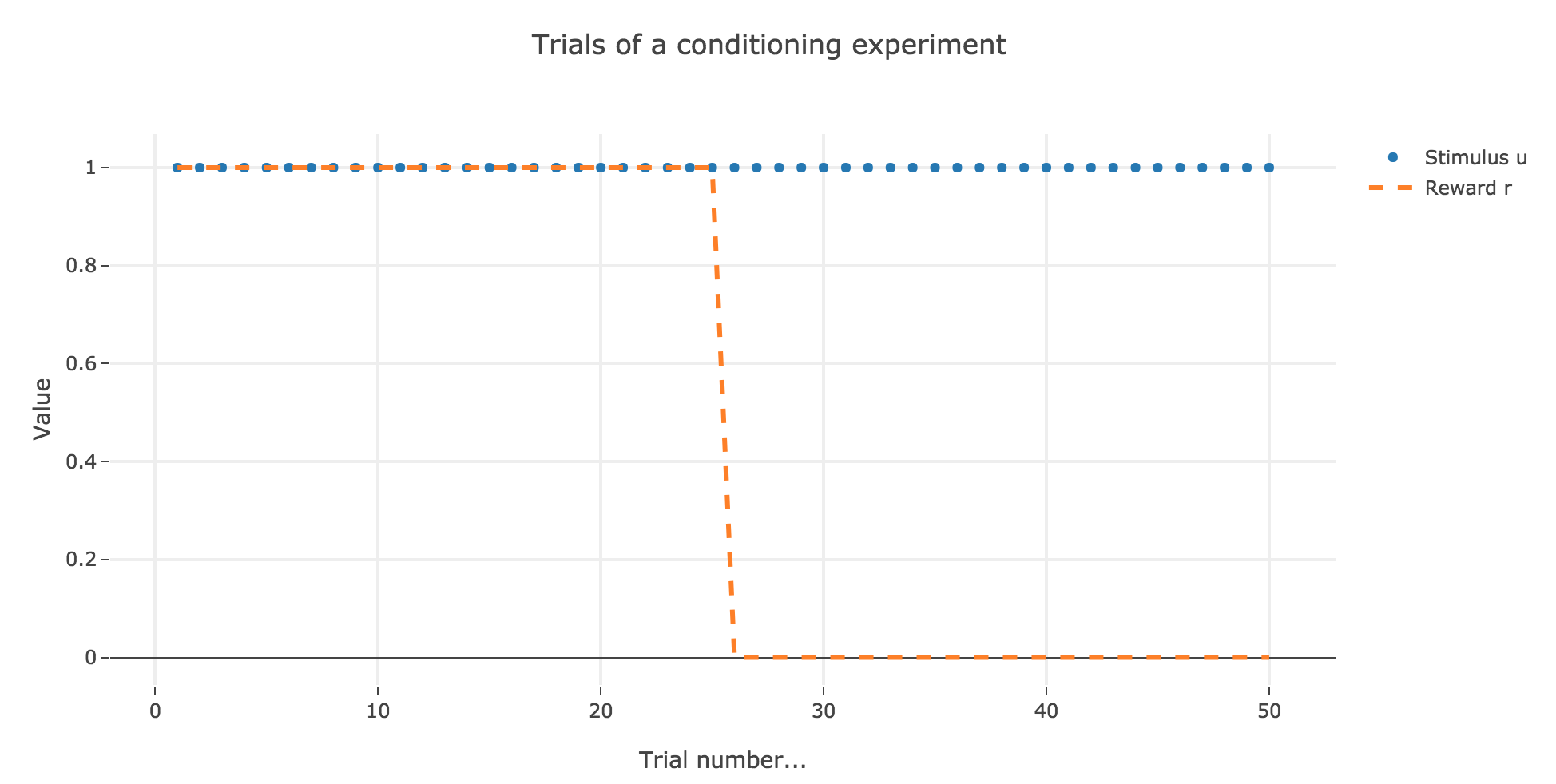Figure 1. - Introductory example: plot of stimulus and reward as a function of the trial index

In which case, according to the Rescola-Wagner rule, the animal prediction $v_i$ is as follows: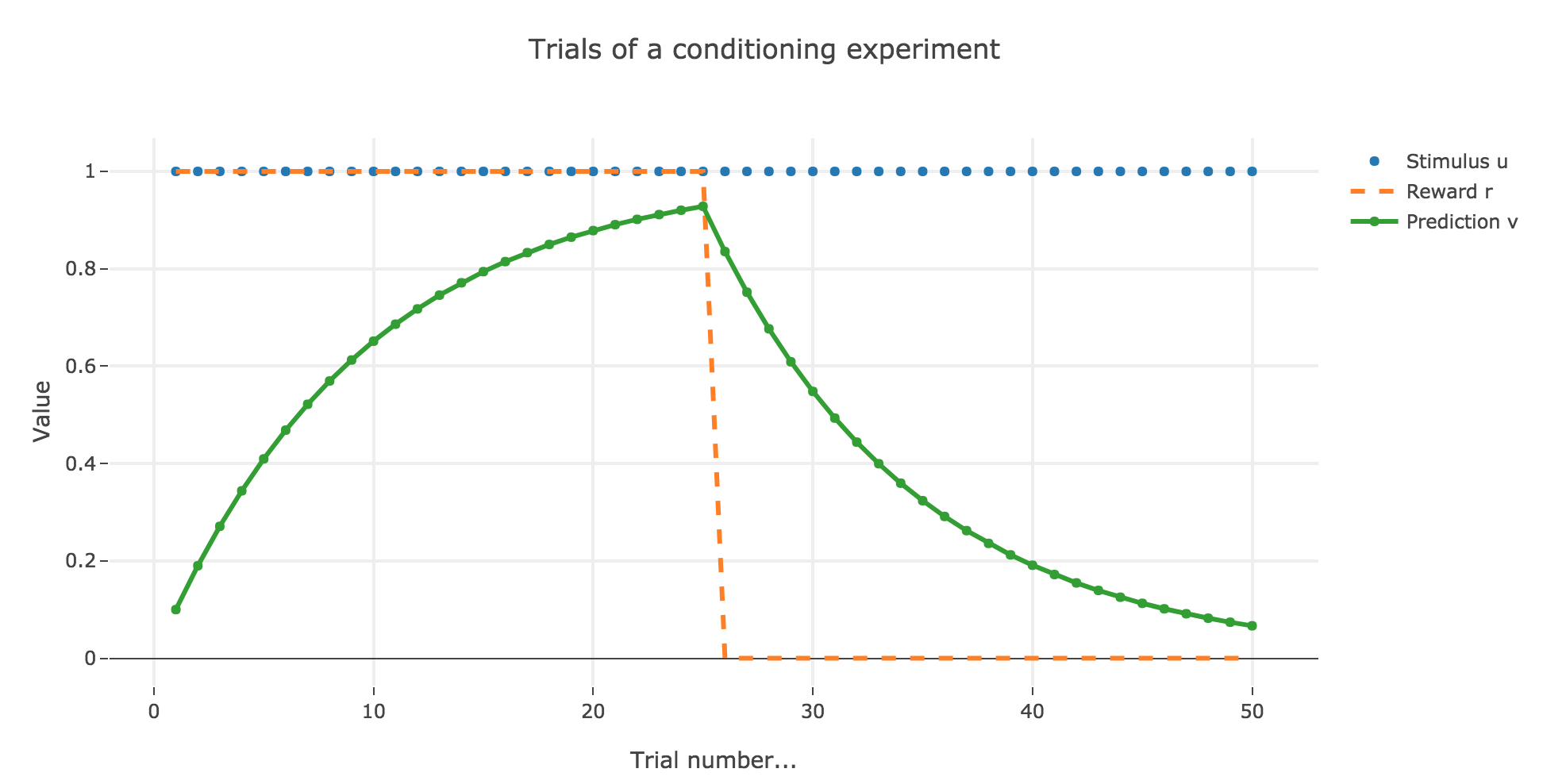Figure 1.2. - Introductory example: plot of the prediction $v$, with $ε = 0.1$ as a function of the trial index

where the learning rate $ε$ is set to be $0.1$.

It appears that the animal prediction $v = wu$ does tend toward the reward. Indeed, if $r_i ≝ r_0$ and $u_i ≝ u_0$ are constant (as it happens here for the first $25$ trials, and for the last $25$ ones):

\begin{align*} w_{i+1} & = w_i + ε (r_0 − u_0 w_i) u_0 \\ & = (1- ε u_0^2) w_i + ε r_0 u_0 \\ \end{align*}

Therefore:

\begin{align*} ∀i ≥ i_0, \; w_i &= (1- ε u_0^2)^{i-i_0} \Big(w_{i_0} - \frac{ε r_0 u_0}{1-(1-ε u_0^2)}\Big) + \frac{ε r_0 u_0}{1-(1-ε u_0^2)}\\ &= \frac{r_0}{u_0}\Big(1 - (1-ε u_0^2)^{i-i_0}\Big) + w_{i_0} (1-ε u_0^2)^{i-i_0} \\ \end{align*}

As a result:

• for the first $25$ trials: $r_0 = u_0 = 1$ and $w_0 = i_0 = 0$, so that:

$∀ i ∈ \lbrace 0, 24 \rbrace, \; w_i = 1 - (1-ε)^i \xrightarrow[i \to +∞]{} 1 = r_0$
• for the last $25$ trials: $r_0 = 0, \; u_0 = 1$ and $i_0 = 24, \, w_{24} > 0$, so that:

$∀ i ∈ \lbrace 25, 50 \rbrace, \; w_i = w_{24} (1-ε)^{i-24} \xrightarrow[i \to +∞]{} 0 = r_0$

### Changing the learning parameter $ε$

Now, let us change the learning rate $ε$ in the previous example.

#### For $ε ≤ 1$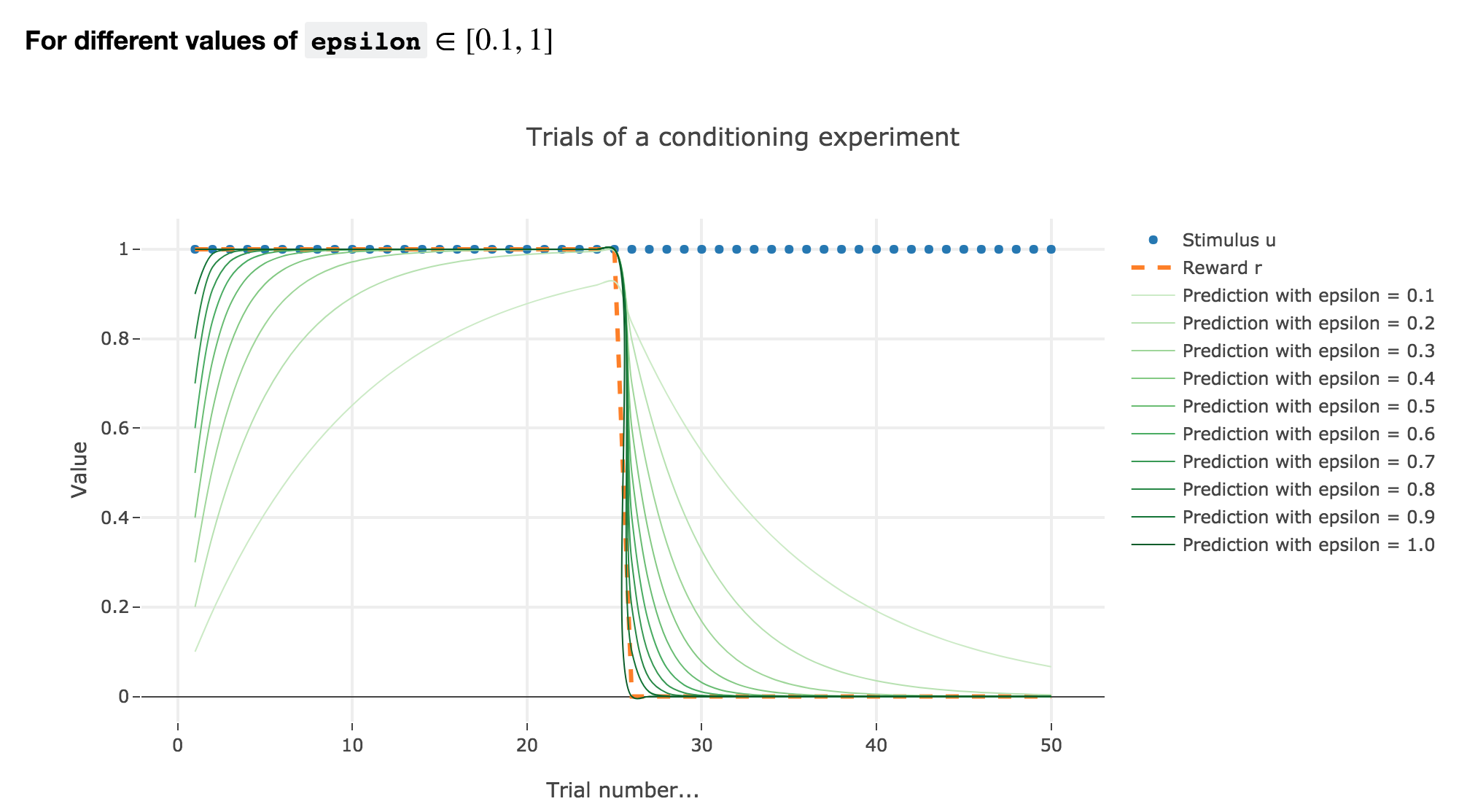Figure 1.3. - Introductory example: plot of the prediction $v$ as a function of the trial index for different values of $0.1 ≤ ε ≤ 1$

As illustrated by the figure above, when $ε ≤ 1$: the bigger the learning rate $ε$, the faster the animal prediction converges toward the reward.

Indeed, as shown above:

$\begin{cases} ∀ i ∈ \lbrace 0, 24 \rbrace, \; w_i = 1 - (1-ε)^i \\ ∀ i ∈ \lbrace 25, 50 \rbrace, \; w_i = w_{24} (1-ε)^{i-24} \end{cases}$

so the bigger the learning rate $ε$, the lower the term $γ ≝ (1-ε)$, and the faster

• $w_i = 1 - γ^i$ converges toward the reward $1$ for the first $25$ trials
• $w_i = w_{24} γ^{i-24}$ converges toward the reward $0$ for the last $25$ trials

It can easily be shown that this observation holds for any constant piecewise reward.

#### For $ε ∈ [1, 2[$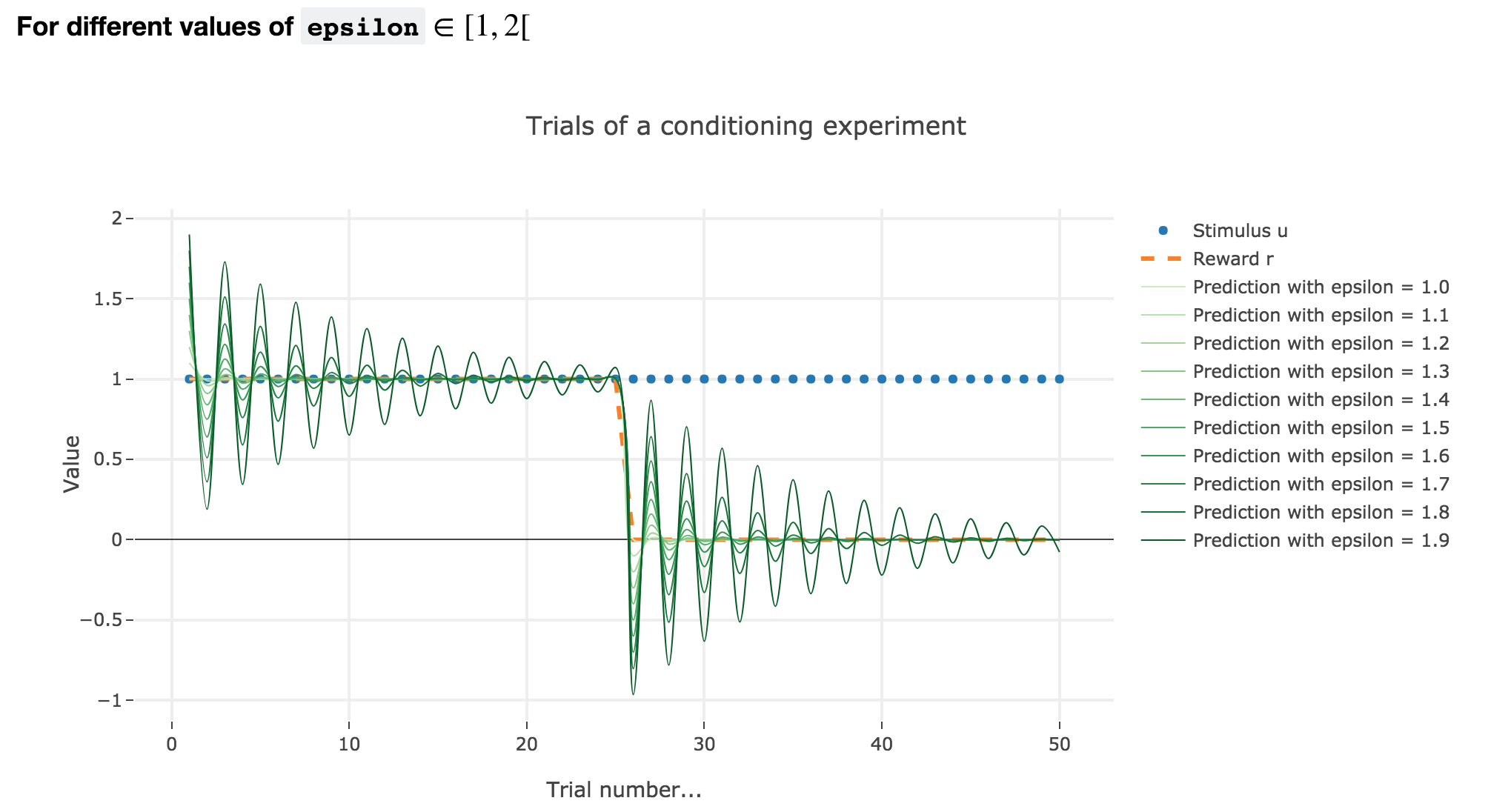Figure 1.4. - Introductory example: plot of the prediction $v$ as a function of the trial index for different values of $1 ≤ ε < 2$

Analogously, if $ε ∈ [1, 2[$: the term $γ ≝ (1-ε) ∈ ]-1, 0]$, and for the first (resp. last) $25$ trials: $w_i = 1 - γ^i$ (resp. $w_i = w_{24} γ^{i-24}$) converges toward the reward $1$ (resp. $0$) while oscillating around it (since $γ ≤ 0$). The oscillation amplitude is all the more significant that $\vert γ \vert$ is big, i.e. that $ε$ is big.

Again, this result can be extended to any constant piecewise reward.

#### For $ε ≥ 2$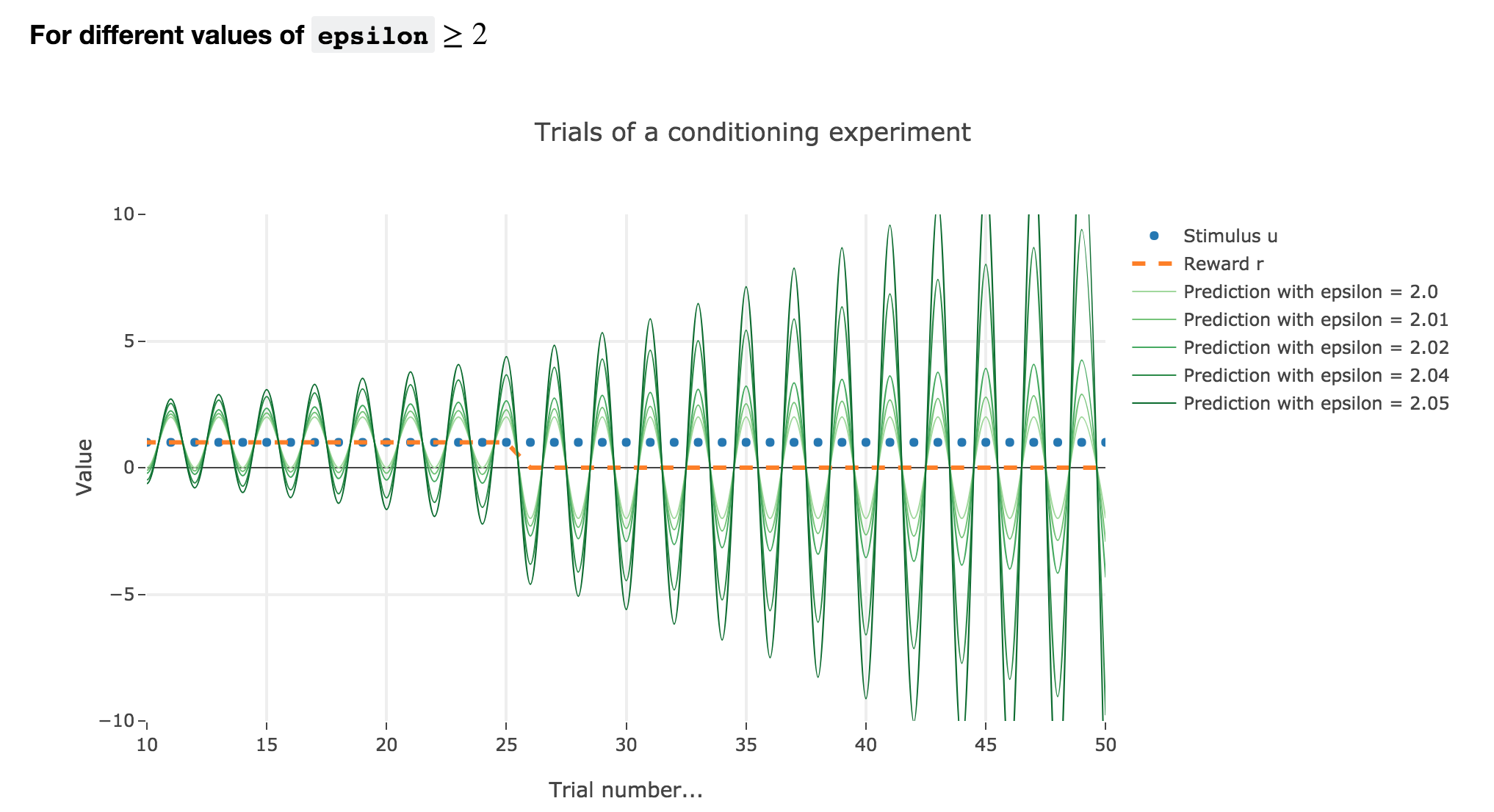Figure 1.5. - Introductory example: plot of the prediction $v$ as a function of the trial index for different values of $ε ≥ 2$

Finally, if $ε ≥ 2$: the term $γ ≝ (1-ε) ≤ -1$, and $w_i$ diverges as $γ^i$ does, while oscillating around it (since $γ ≤ 0$). The oscillation amplitude is all the more significant that $\vert γ \vert$ is big, i.e. that $ε$ is big.

Again, this result stands for any constant piecewise reward.

Going back to our conditioning experiment:

• $ε ≤ 1$ corresponds to what is expected from the experiments: the bigger the learning rate, the faster the convergence of the animal prediction, monotonously for a constant reward: hence the name learning rate.

• $ε ∈ [1 , 2[$ and $ε ≥ 2$ are both degenerate cases: $ε ≥ 2$ is completely nonsensical from a biological standpoint, and the oscillations of $ε ∈ [1 , 2[$ are not what we want to model, which is rather a convergence of the animal prediction straight to the reward.

## 2. Partial conditioning

Let us suppose that in each trial, the stimulus is present, but the presence of the reward is a random event with probability $0.4$.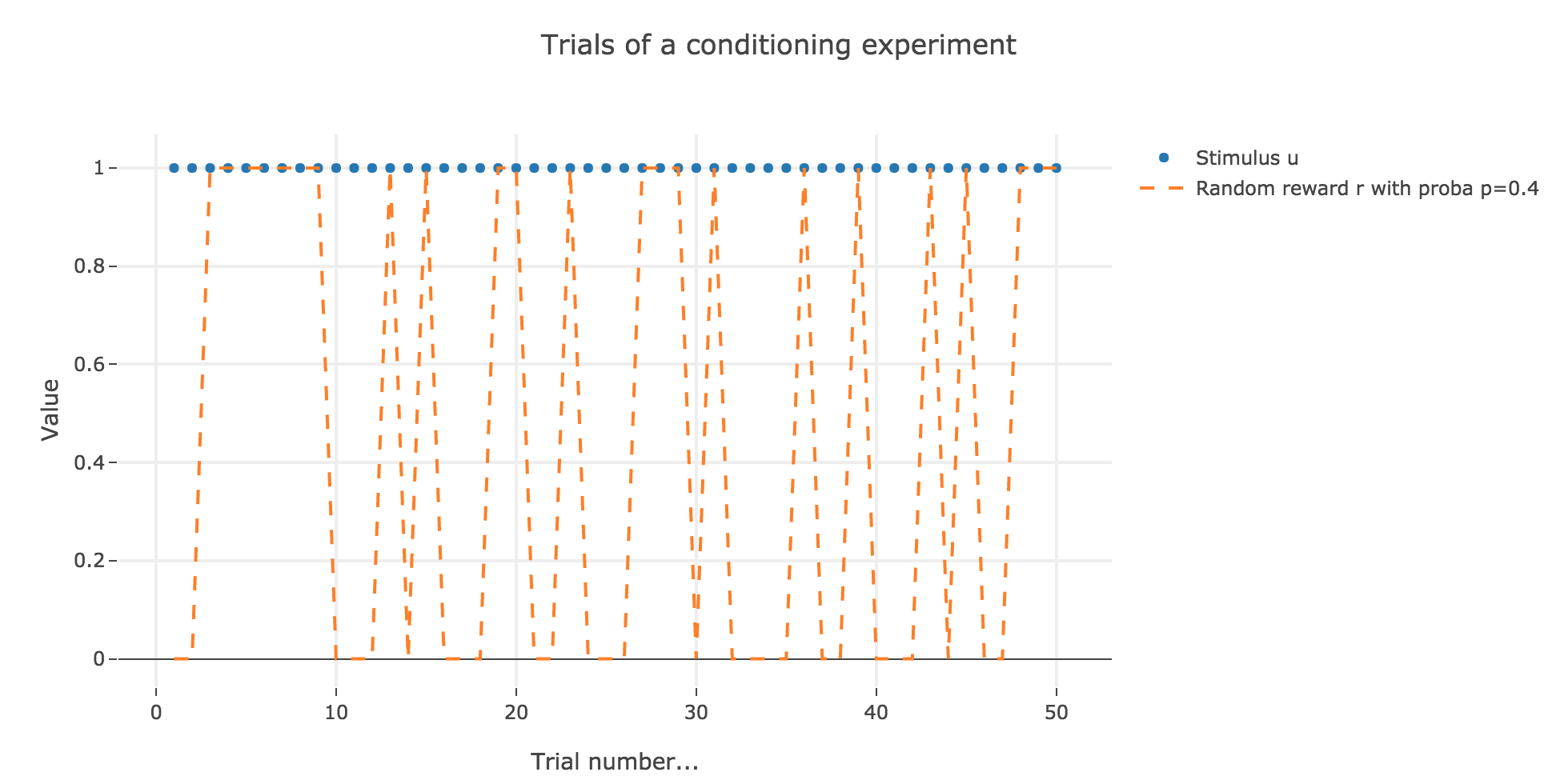Figure 2.1. - Partial conditioning: plot of the reward as a function of the trial index

Similarly to what has been done in the previous section: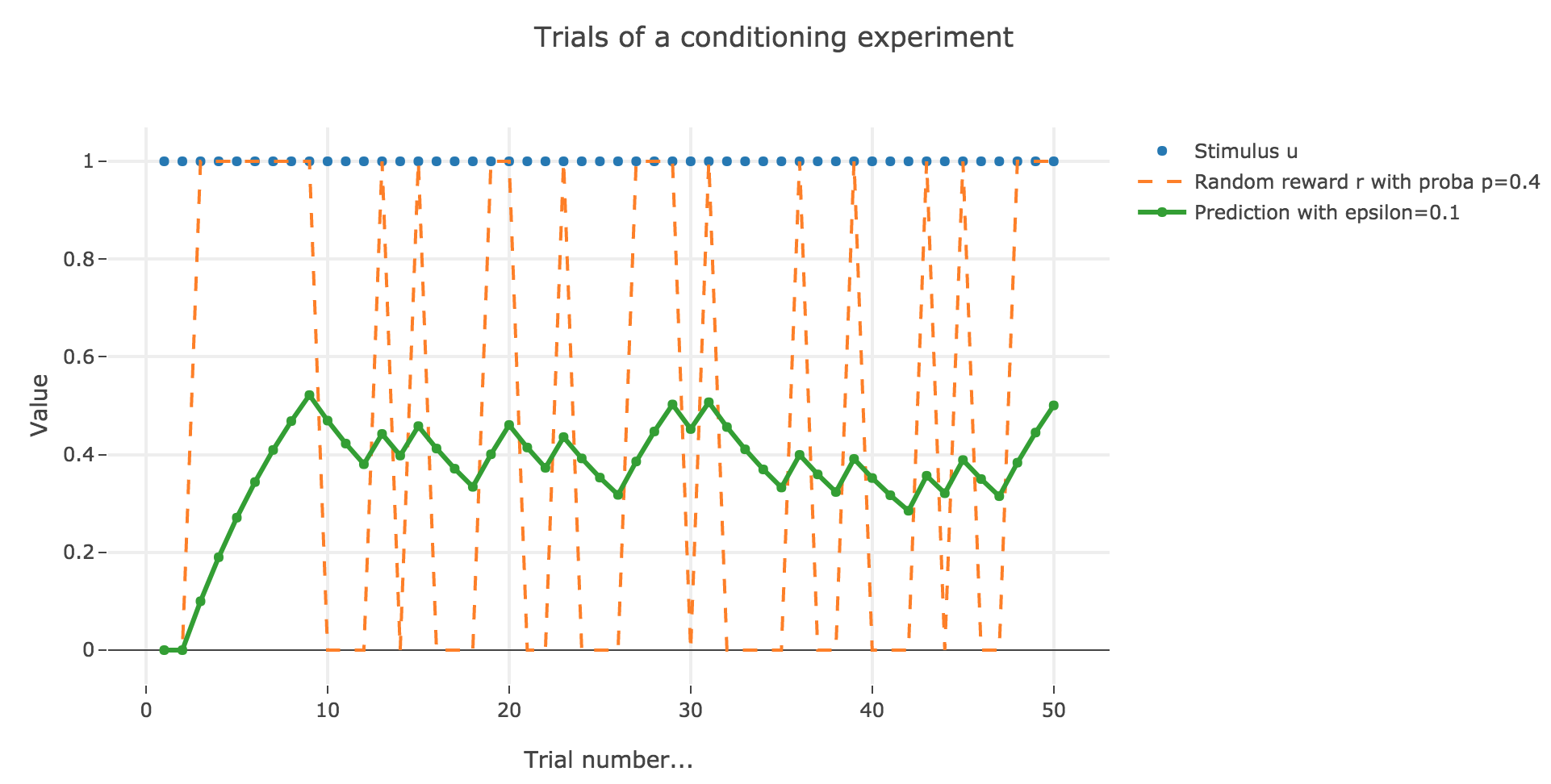Figure 2.2. - Partial conditioning: plot of the animal prediction as a function of the trial index, for $ε = 0.1$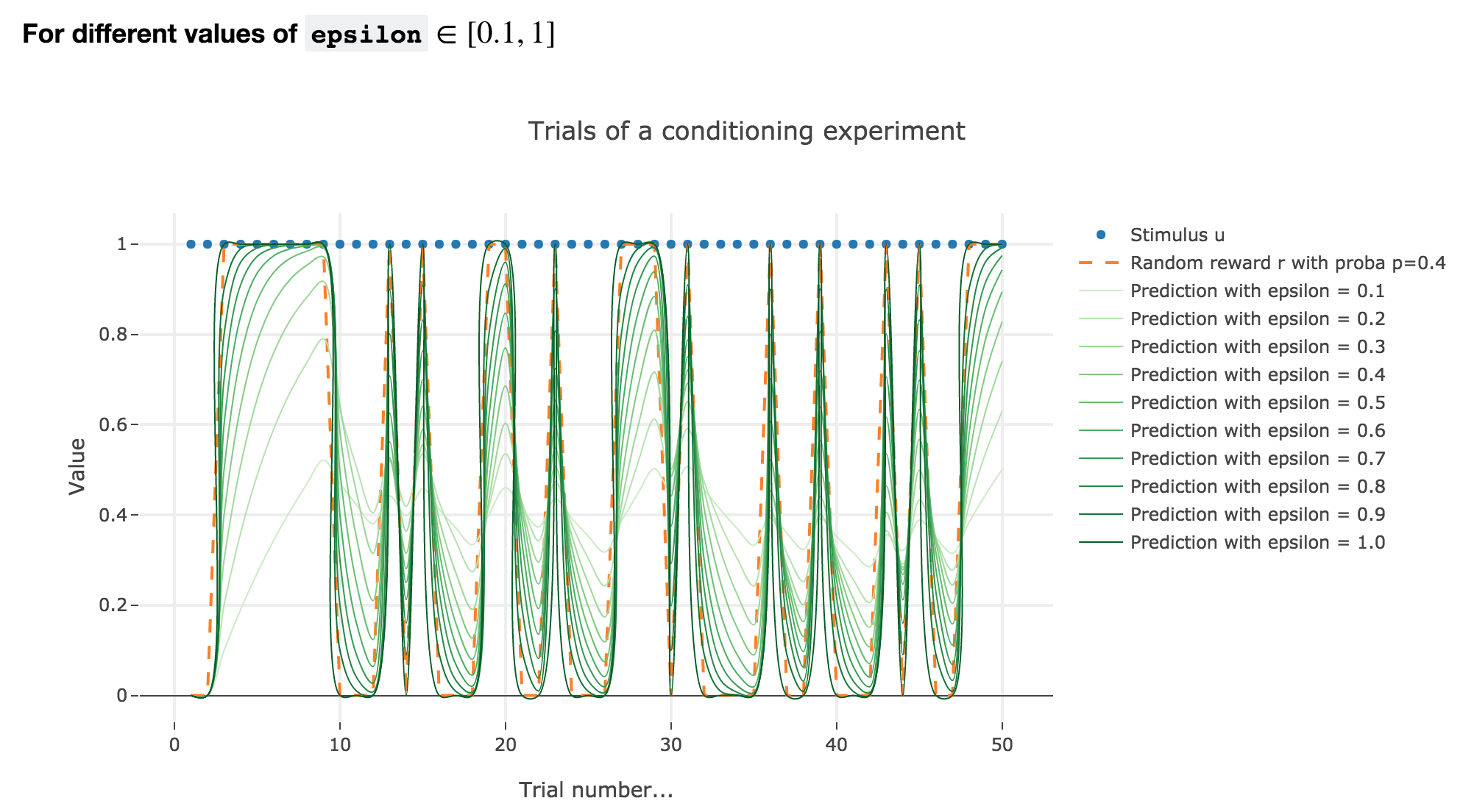Figure 2.3. - Partial conditioning: plot of the animal prediction as a function of the trial index for different values of $ε ∈ [0.1, 1]$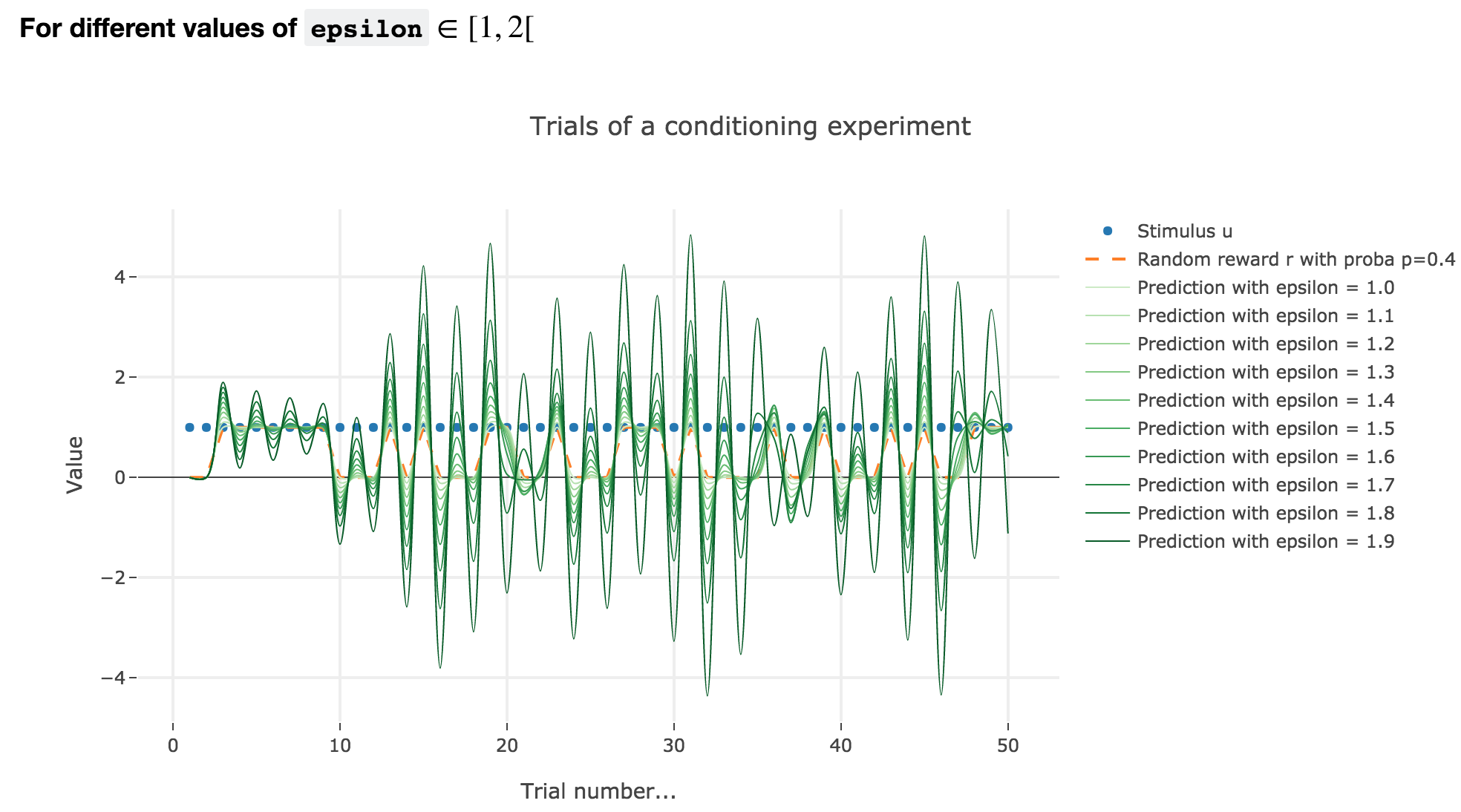Figure 2.4. - Partial conditioning: plot of the animal prediction as a function of the trial index for different values of $ε ∈ [1, 2[$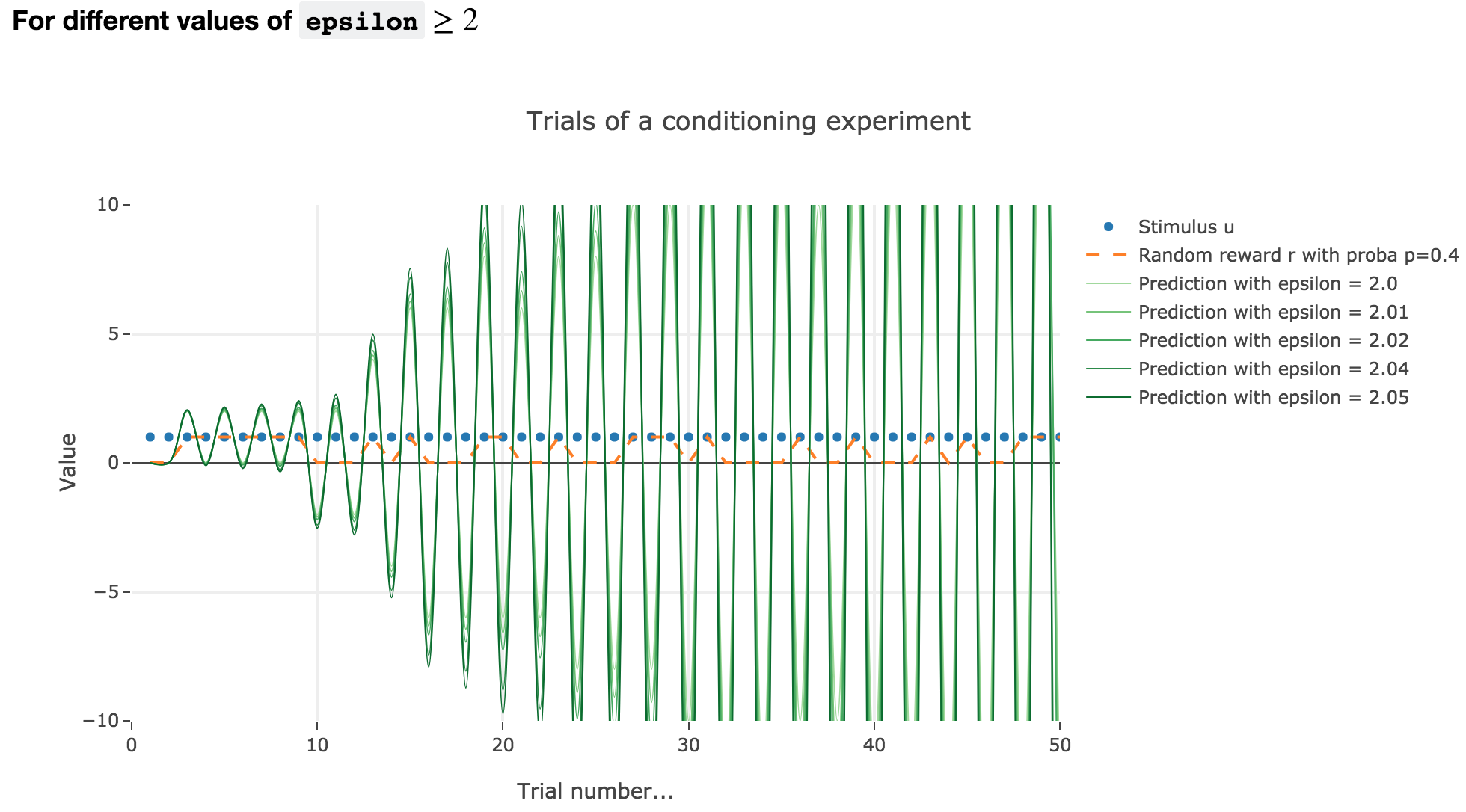Figure 2.5. - Partial conditioning: plot of the animal prediction as a function of the trial index for different values of $ε ≥ 2$

As it happens, the reward is a constant piecewise function, so the previous analysis applies here, by focusing on each segment where the reward is constant (the stimulus is always equal to $1$ here, which simplifies things up). On top of that, we may stress that the shorter these segments are, the higher $ε ≤ 1$ has to be, for the animal to learn the reward within these shorter time spans. The oscillating cases are still irrelevant from a biological standpoint.

## 3. Blocking

Now, we assume that there are two stimuli, $u_1$ and $u_2$, and two parameters, $w_1$ and $w_2$ to learn, and that the animal prediction is given by $v = w_1 u_1 + w_2 u_2$.

During the first $25$ trials, only one stimulus and the reward are present, during the next $25$ trials, both stimuli and the reward are present.

For the record, here is what happens when there is one single stimulus and $u = r = 1$: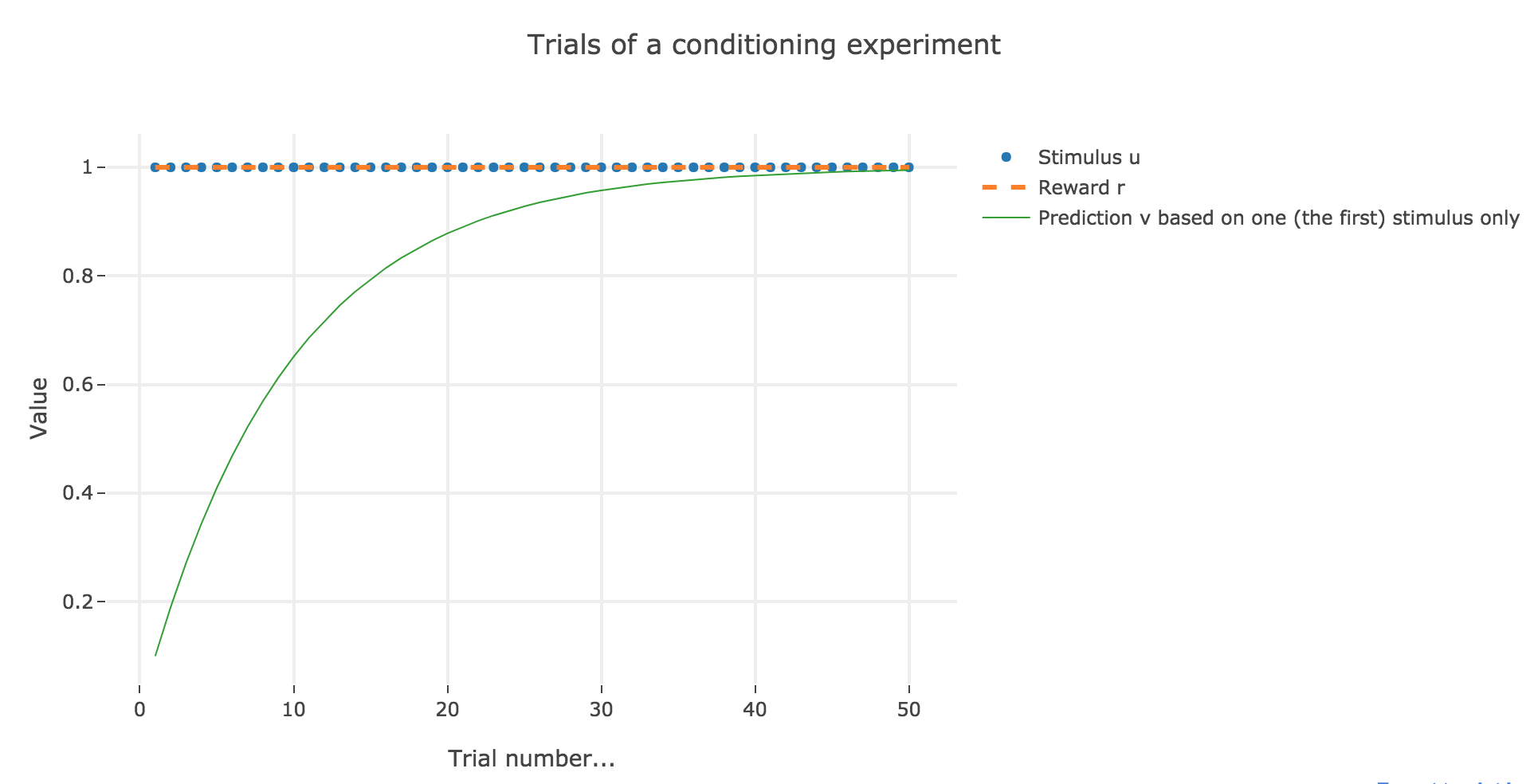Figure 3.1. - Plot of the animal prediction as a function of the trial index for one single stimulus ($ε = 0.1$)

Now, with aforementioned two stimuli: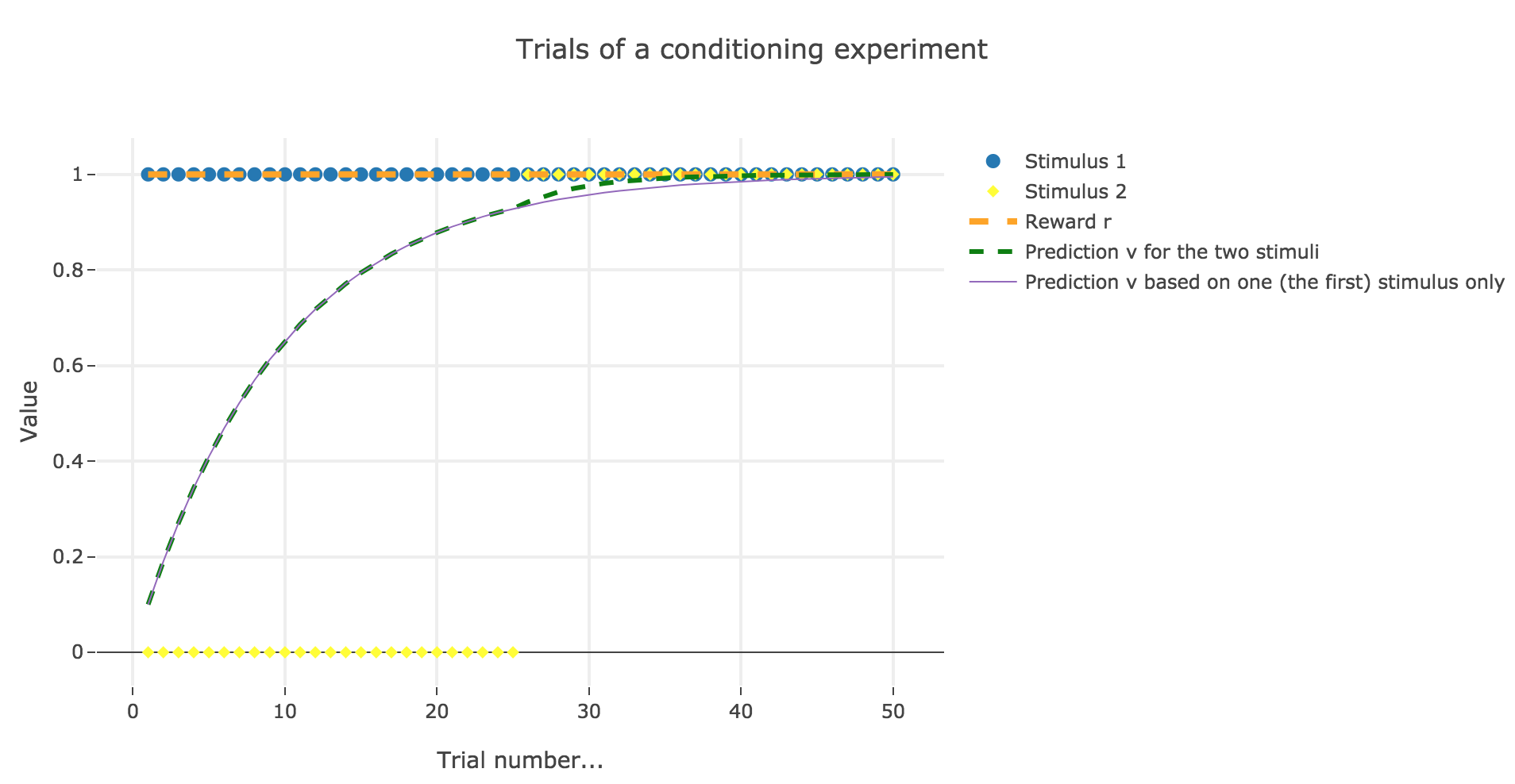Figure 3.2. - Plot of the animal prediction for two stimuli $u_1, u_2$ and one single stimulus $u_1$ ($ε = 0.1$)

To be more precise, here is the evolution of $w_1$ and $w_2$ throughout the trials:

Trial $w_1$ $w_2$
1 0.1 0.
2 0.19 0.
3 0.271 0.
4 0.3439 0.
5 0.40951 0.
6 0.468559 0.
7 0.5217031 0.
8 0.56953279 0.
9 0.61257951 0.
10 0.65132156 0.
11 0.6861894 0.
12 0.71757046 0.
13 0.74581342 0.
14 0.77123208 0.
15 0.79410887 0.
16 0.81469798 0.
17 0.83322818 0.
18 0.84990536 0.
19 0.86491483 0.
20 0.87842335 0.
21 0.89058101 0.
22 0.90152291 0.
23 0.91137062 0.
24 0.92023356 0.
25 0.9282102 0.
26 0.93538918 0.00717898
27 0.94113237 0.01292216
28 0.94572691 0.01751671
29 0.94940255 0.02119235
30 0.95234306 0.02413286
31 0.95469547 0.02648527
32 0.95657739 0.02836719
33 0.95808294 0.02987273
34 0.95928737 0.03107717
35 0.96025092 0.03204071
36 0.96102175 0.03281155
37 0.96163842 0.03342822
38 0.96213176 0.03392156
39 0.96252643 0.03431623
40 0.96284216 0.03463196
41 0.96309475 0.03488455
42 0.96329682 0.03508662
43 0.96345848 0.03524827
44 0.9635878 0.0353776
45 0.96369126 0.03548106
46 0.96377403 0.03556383
47 0.96384024 0.03563004
48 0.96389321 0.03568301
49 0.96393559 0.03572539
50 0.96396949 0.03575929

There is a phenomenon of blocking in the sense that: when $u_2$ appears (from the trial $26$ on), the prediction error is already close to zero (the animal has already almost exclusively associated the presence of the reward with the presence of the first stimulus only), so that the second stimulus is hardly taken into account, compared to the first one (hence the blocking).

We still suppose that there are two stimuli and two parameters to learn. However, now:

• both stimuli and the reward are present throughout all the trials.

• one of the learning rates is larger: $ε_1 = 0.2$ for the first stimulus, and $ε_2 = 0.1$ for the other one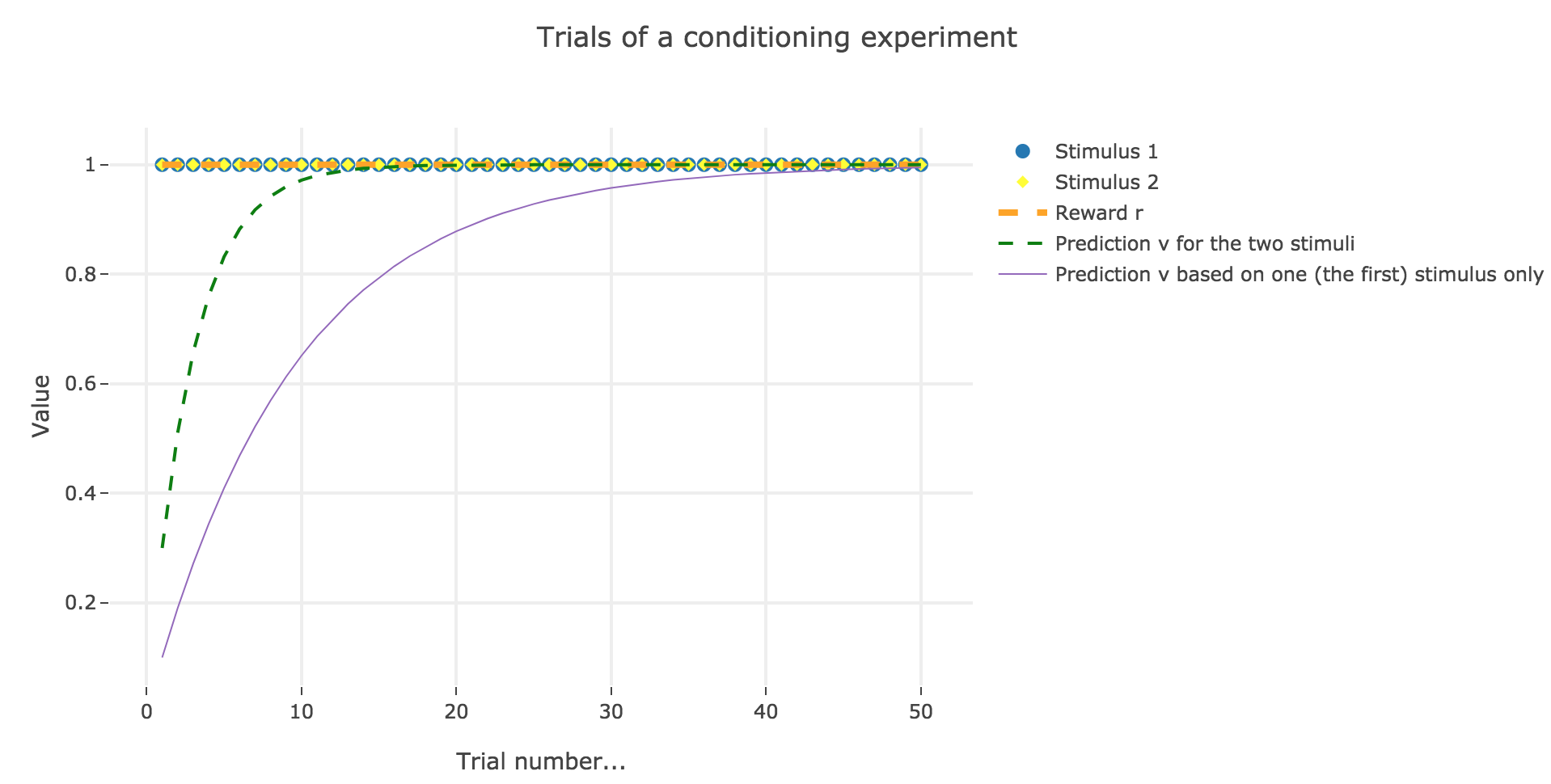Figure 4.1. - Plot of the animal prediction for two stimuli $u_1, u_2$ (with $ε_1 = 0.2, ε_2 = 0.1$) and one single stimulus $u_1$ (with $ε = 0.1$)

Here is the evolution of $w_1$ and $w_2$ throughout the trials:

Trial $w_1$ $w_2$
1 0.2 0.1
2 0.34 0.17
3 0.438 0.219
4 0.5066 0.2533
5 0.55462 0.27731
6 0.588234 0.294117
7 0.6117638 0.3058819
8 0.62823466 0.31411733
9 0.63976426 0.31988213
10 0.64783498 0.32391749
11 0.65348449 0.32674224
12 0.65743914 0.32871957
13 0.6602074 0.3301037
14 0.66214518 0.33107259
15 0.66350163 0.33175081
16 0.66445114 0.33222557
17 0.6651158 0.3325579
18 0.66558106 0.33279053
19 0.66590674 0.33295337
20 0.66613472 0.33306736
21 0.6662943 0.33314715
22 0.66640601 0.33320301
23 0.66648421 0.3332421
24 0.66653895 0.33326947
25 0.66657726 0.33328863
26 0.66660408 0.33330204
27 0.66662286 0.33331143
28 0.666636 0.333318
29 0.6666452 0.3333226
30 0.66665164 0.33332582
31 0.66665615 0.33332807
32 0.6666593 0.33332965
33 0.66666151 0.33333076
34 0.66666306 0.33333153
35 0.66666414 0.33333207
36 0.6666649 0.33333245
37 0.66666543 0.33333271
38 0.6666658 0.3333329
39 0.66666606 0.33333303
40 0.66666624 0.33333312
41 0.66666637 0.33333318
42 0.66666646 0.33333323
43 0.66666652 0.33333326
44 0.66666656 0.33333328
45 0.6666666 0.3333333
46 0.66666662 0.33333331
47 0.66666663 0.33333332
48 0.66666664 0.33333332
49 0.66666665 0.33333332
50 0.66666665 0.33333333

There is a phenomemon of overshadowing: the first stimulus ends up being taken into account twice as much as the second (overshadowing it), as it has been learned at a rate two times bigger.

# PROBLEM 2: Simple decision strategy for flower sampling by bees.

Link of the iPython notebook for the code

Let us consider the following experiment: a bee lands on flowers to collect as much nectar as possible, during two day (granting that it lands on $100$ flowers a day). There are two kinds of flowers:

• blue flowers, which carry a nectar reward of

• $r_b = 8$ during the first day
• $r_b = 2$ during the second day
• yellow flowers, which carry a nectar reward of

• $r_y = 2$ during the first day
• $r_y = 8$ during the second day

The bee’s internal estimate for the rewards is $m_b$ (resp. $m_y$) for the blue (resp. yellow) flowers. The bee chooses to land on a blue flower with probability (softmax-strategy):

$p_b = \frac{1}{1 + \exp(β (m_y - m_b))}$

where $β$ is called the “exploitation-exploration trade-off” parameter (we will explain why in the following section).

## 1. The softmax-strategy

Note that $p_b$ is a sigmoid of $-(m_y - m_b)$ (for a fixed $β$) and a sigmoid of $-β$ (for a fixed $m_y - m_b$).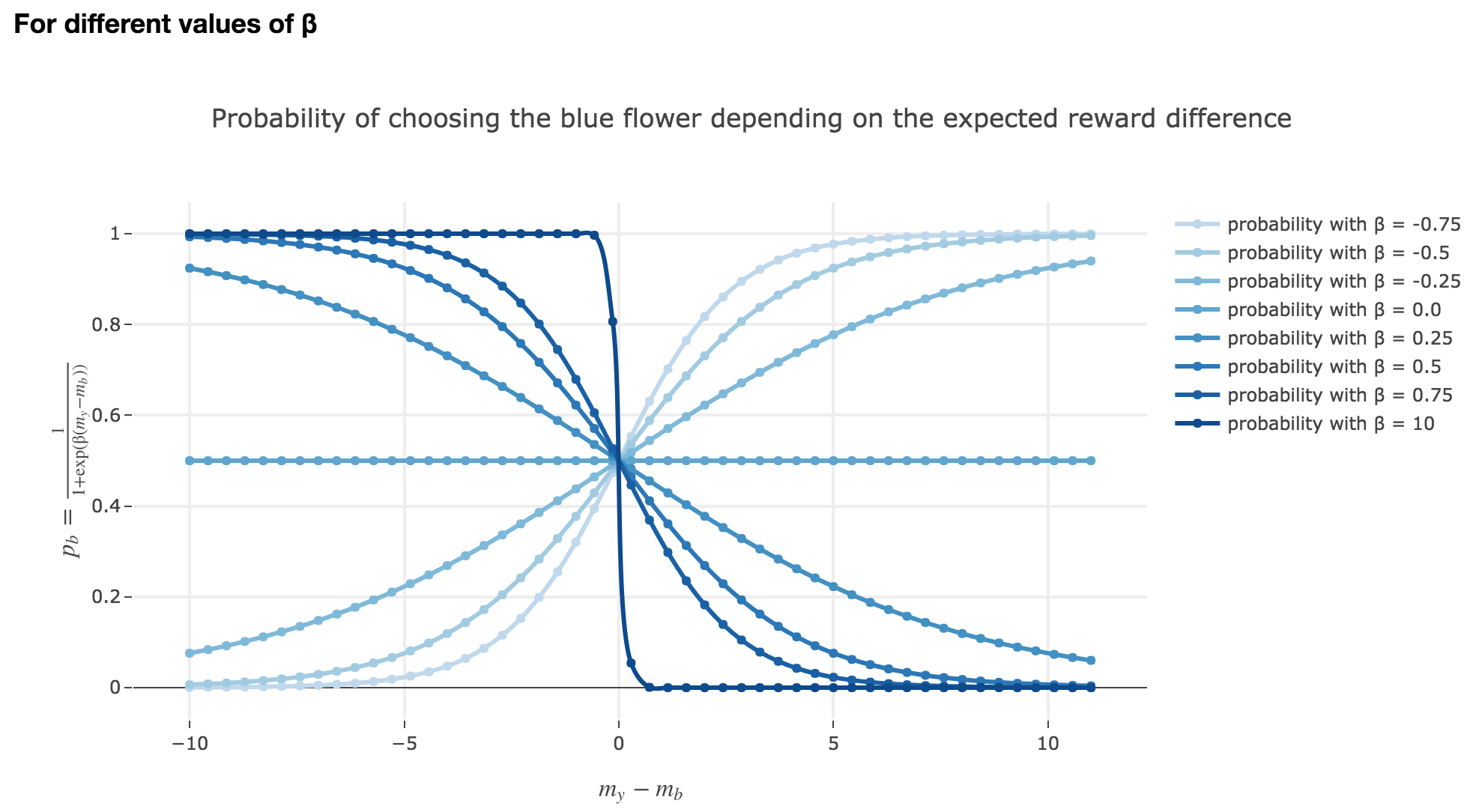Figure 4.1. - Plot of $p_b$ for different values of $β$

Thus:

• the larger $m_y - m_b$, the higher the bee’s internal estimate of the yellow flower is compared to the blue one. As a consequence, the lower the probability $p_b$ of the bee landing on the blue one.

• the lower the reward difference, the worse the bee considers the yellow flower to be, compared to the blue one, and the greater the probability $p_b$ of the bee landing on the blue flower.

• in between: the bee’s behavior is a mix between exploiting what the bee deems to be the most nutritious flower and exploring the other one (which is what is expected).

Symetrically: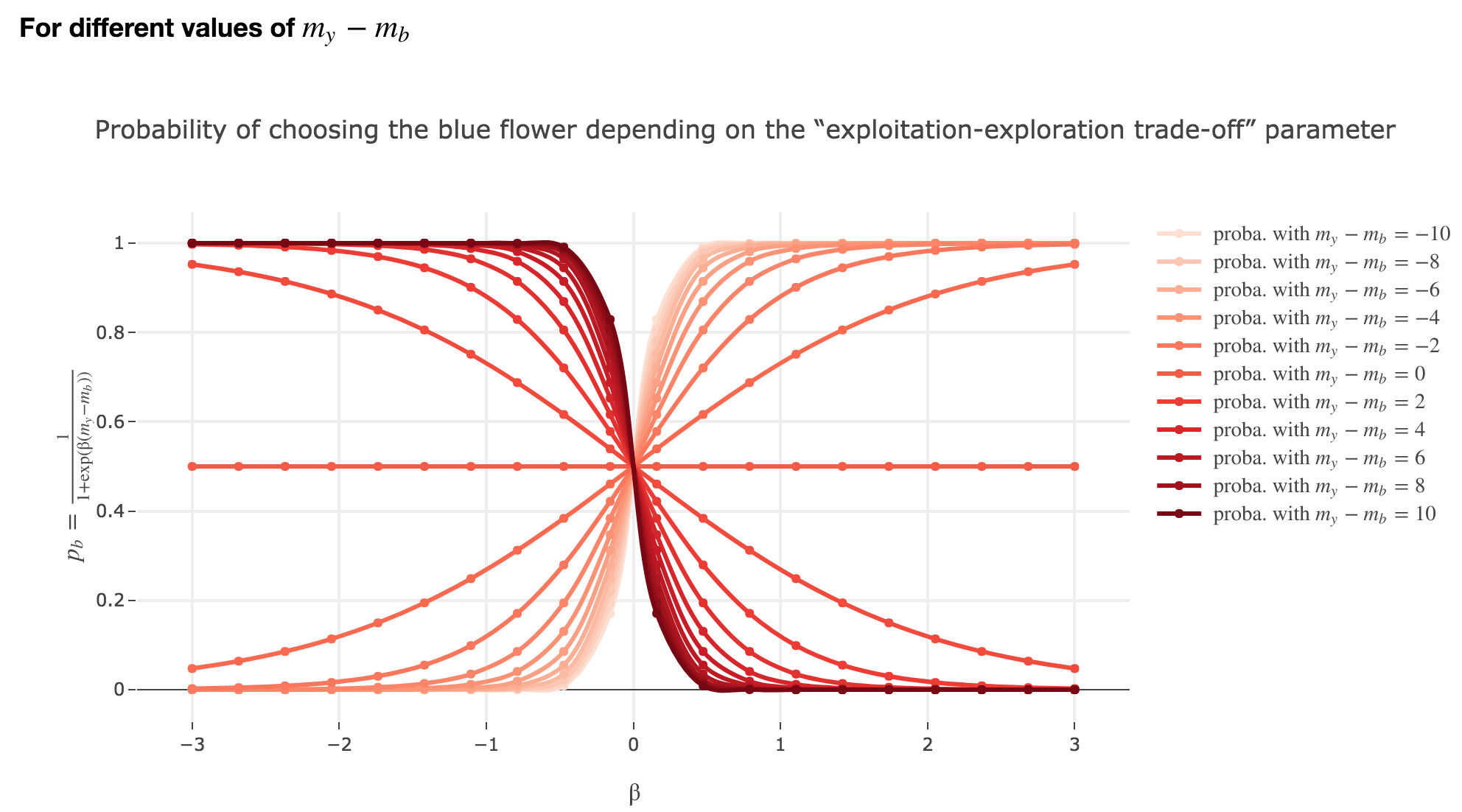Figure 4.1. - Plot of $p_b$ for different values of $m_y-m_b$

### The “exploitation-exploration trade-off” parameter $β$

#### For $β ≥ 0$

As seen in the previous figures: the parameter $β$ plays the same role as the inverse temperature $β ≝ \frac{1}{k_B T}$ in statistical physics. That is, for $β ≥ 0$:

• When $β ⟶ +∞$: the bigger $β$ is (which corresponds, in physics, to a low temperature/entropy), the more the bee tends to exploit the seemingly most nutritious flower. As a result:

• if $m_y-m_b > 0$ (i.e. the yellow flower seems more avantageous to the bee), the probability of the bee landing on the blue flower rapidly decreases to $0$
• if $m_y-m_b < 0$ (i.e. the blue flower seems more advantageous to the bee), the probability of the bee landing on the blue flower rapidly increases to $1$
• When $β ⟶ 0$: the lower $β$ is (high temperature/entropy in physics), the more the bee tends to explore the flowers.

Indeed: as $β ⟶ 0$, $p_b$ becomes less and less steep, to such a point that it ends up being the constant function $1/2$ when $β=0$ (at this point, the bee does nothing but exploring, since landing on either of the flowers is equiprobable, no matter how nutritious the bee deems the flowers to be)

#### For $β < 0$

As

$p_b(-β, m_y-m_b) = \frac{1}{1 + \exp(-β (m_y-m_b))} = \frac{1}{1 + \exp(β (-(m_y-m_b)))} = p_b(β, -(m_y-m_b))$

The curves for $-β < 0$ are symmetric to those for $β > 0$ with respect to the y-axis, which makes no sense from a behavioral point of vue: it means that the most nutritious a flower appears to the bee, the less likely the bee is to land on it (and conversely)!

## 2. Dumb bee

Now, let us assume that the bee is “dumb”, i.e. it does not learn from experience: throughout all the trials, the internal reward estimates remain constant: $m_y = 5, \; m_b = 0$.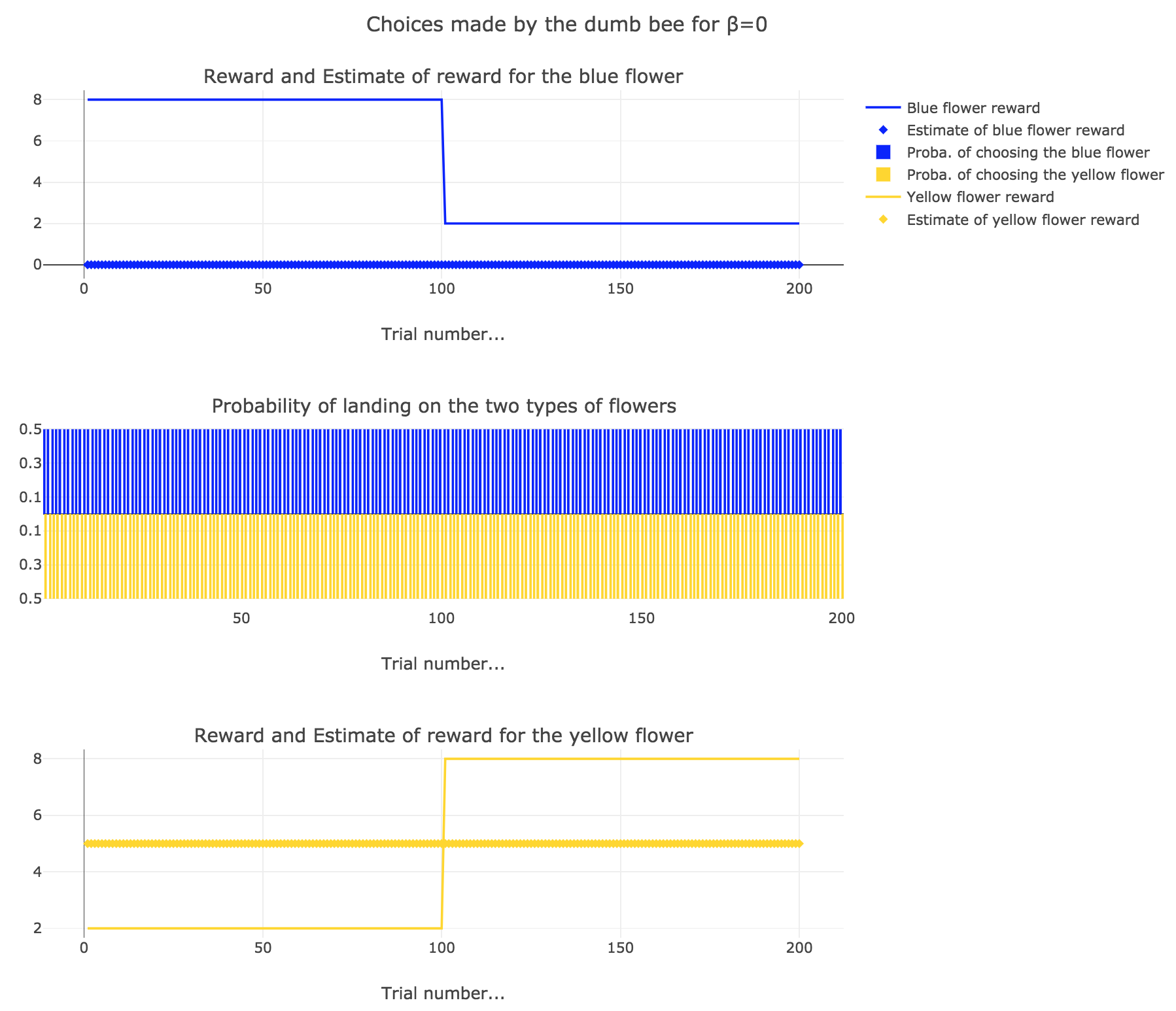Figure 2.1. - Dumb bee: Behavior for $β=0$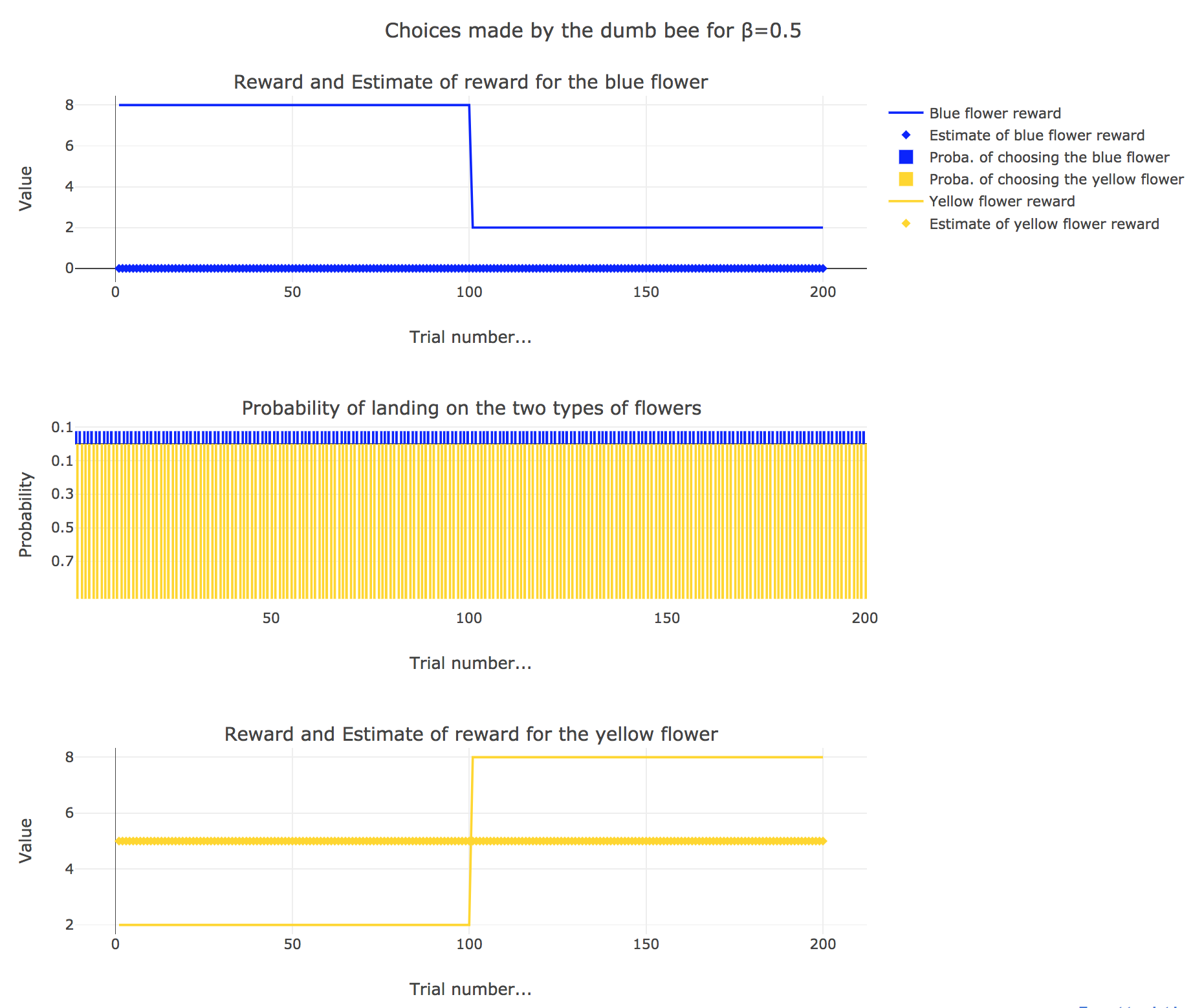Figure 2.2. - Dumb bee: Behavior for $β=0.5$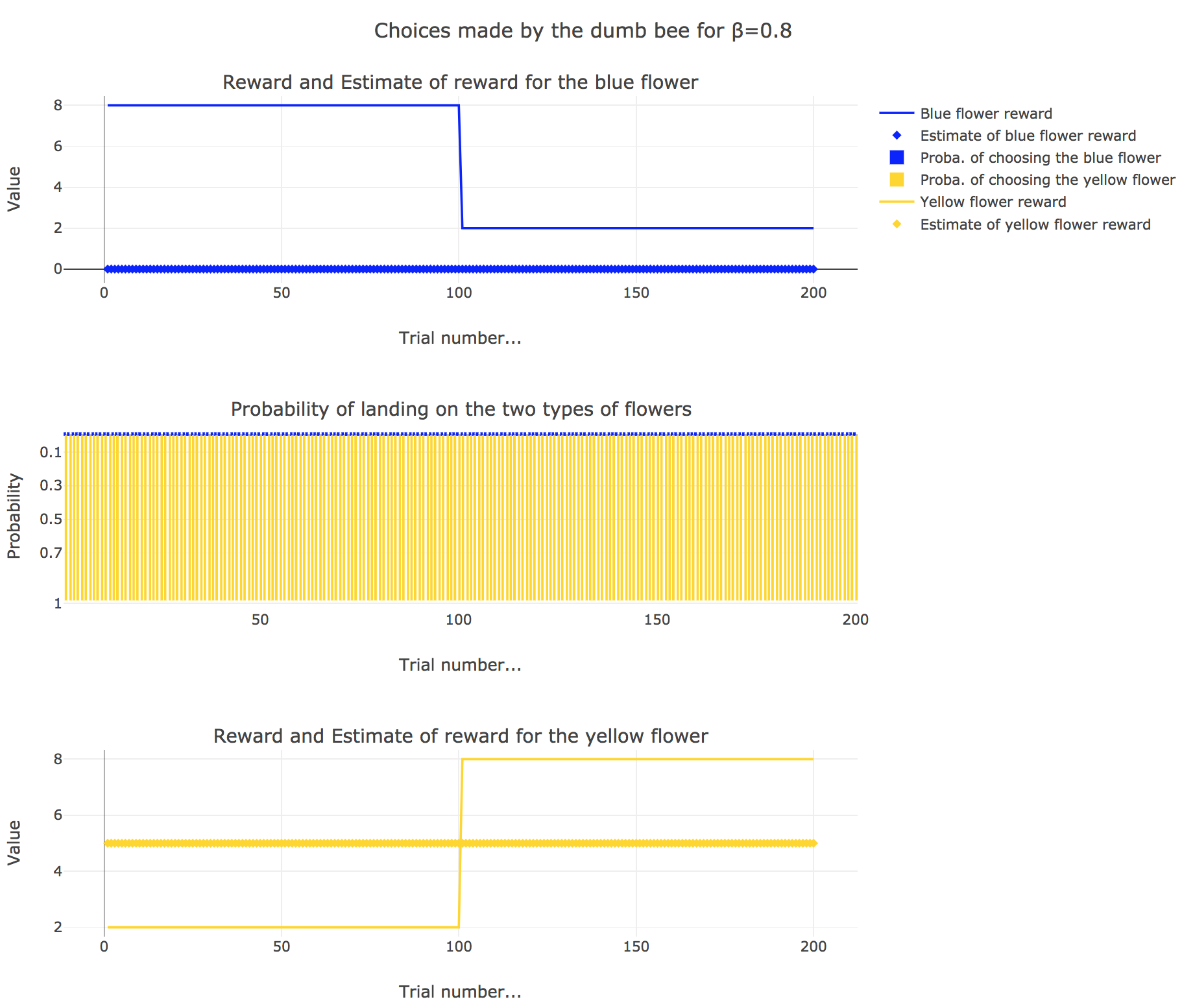Figure 2.3. - Dumb bee: Behavior for $β=1$

So it appears that

• for $β = 0$: the bee’s behavior is the most exploratory one: the probability of landing on a blue flower as well as a yellow flower is $1/2$, irrespective of the internal estimates $m_b$ and $m_y$

• for $β = 0.5$: this $β$ corresponds to a perfectly balanced exploration-exploitation tradoff, but here: as $m_y = 5$ is significantly higher that $m_b = 0$, $p_y ≝ 1-p_b » p_b$ (which are constant, as the internal estimates are constant)

• for $β = 1$: the bee’s behavior is the most exploitative one. As $m_y > m_b$, $p_y ≃ 1$: the bee keep exploiting the flower it deems the most nutritious (i.e. the yellow ones).

On the whole, this behavior can reasonably be called “dumb”, since the bee takes no account of the actual rewards of the flowers whatsoever (its internal estimates remain constant and don’t depend on these actual rewards).

## 3. Smart bee

Now let us suppose that the bee is “smart”, i.e. it can learn from its experiences. Whenever it visits a flower, it updates its estimated reward as follows:

\begin{align*} m_b &→ m_b + ε(r_b − m_b)\\ m_y &→m_y +ε(r_y − m_y) \end{align*}

Given a learning parameter $ε = 0.2$ and the initial assumptions about flower reward from above ($m_y = 5, m_b = 0$), simulate the bees sequence of choices during the two days. How do the reward estimates change over time? Explore the case of purely explorative behavior ($β = 0$) and the case of strongly exploitative behavior ($β = 1$). What do you observe?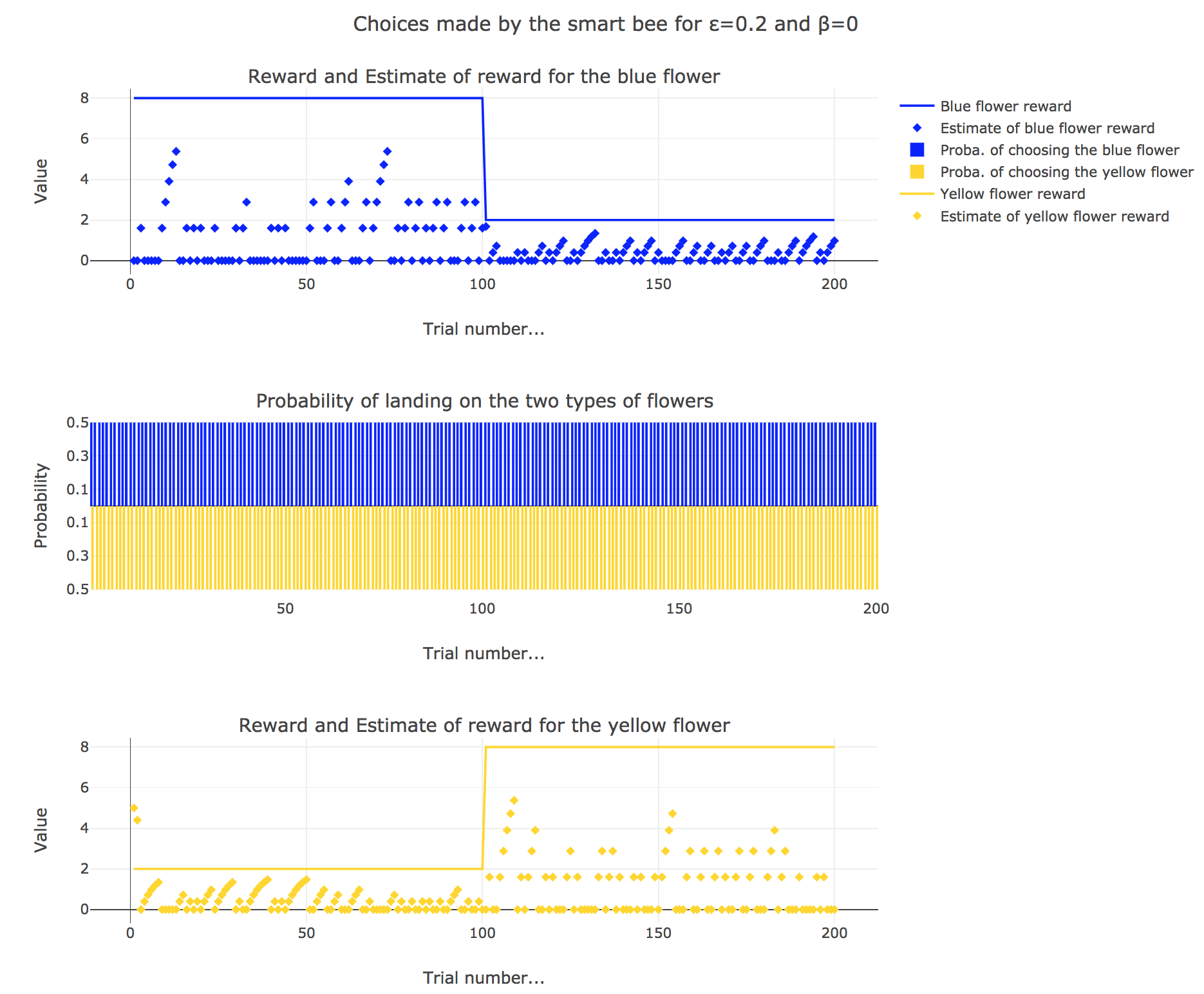Figure 3.1. - Smart bee: Behavior for $β=0$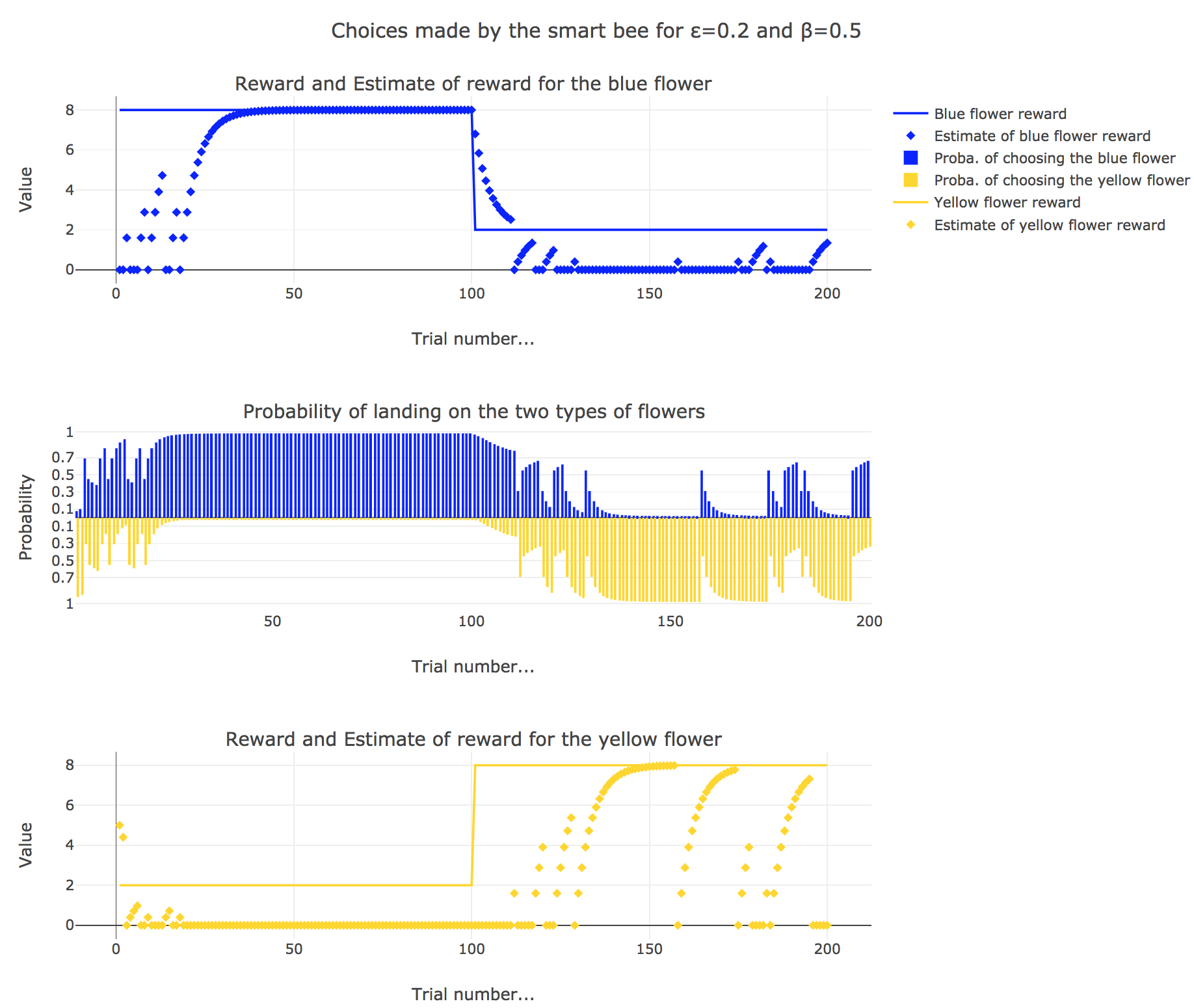Figure 3.2. - Smart bee: Behavior for $β=0.5$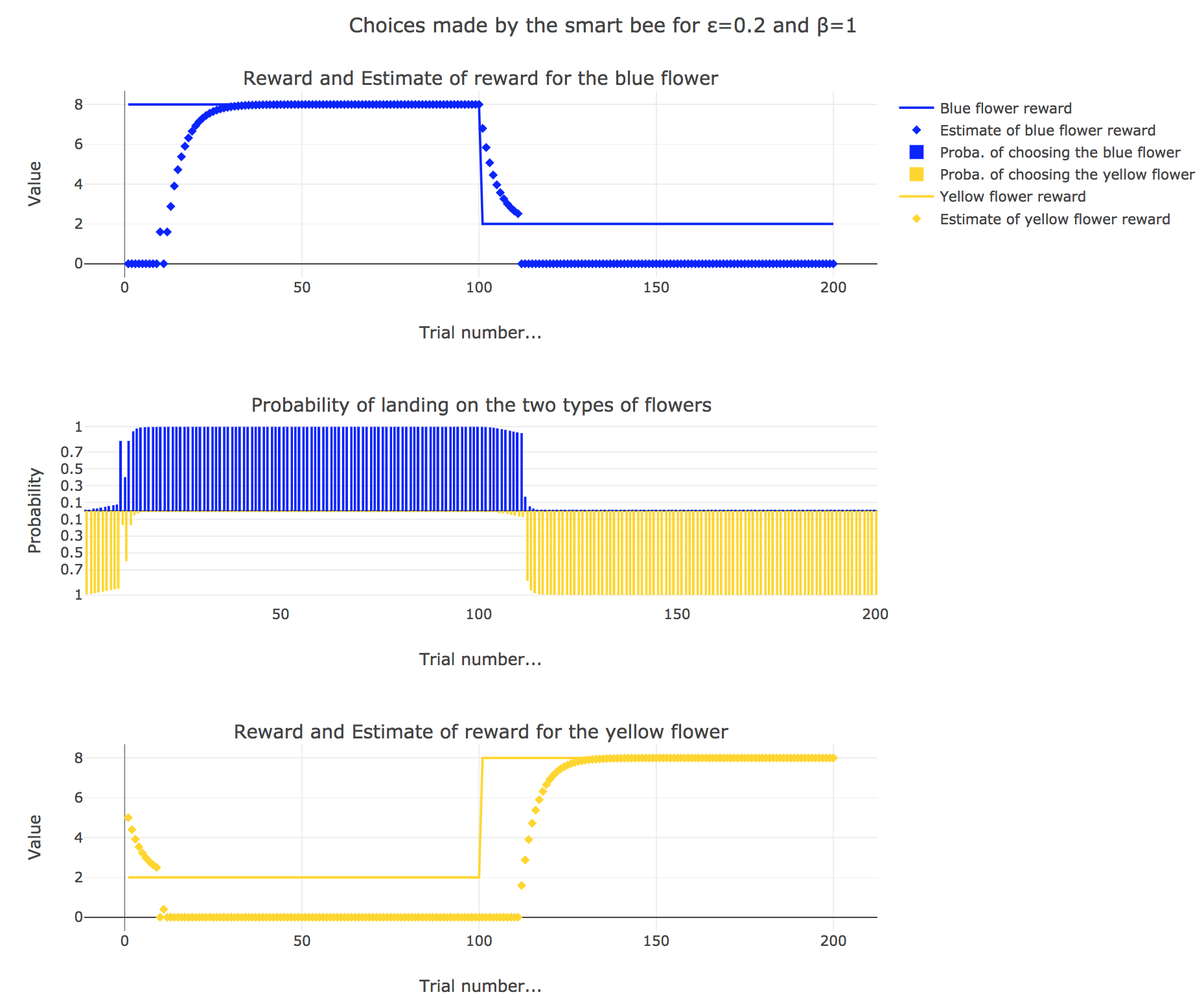Figure 3.3. - Smart bee: Behavior for $β=1$

It appears that

• for $β = 0$: no surprise, the bee’s behavior is the most exploratory one: the probability of landing on a blue flower as well as a yellow flower is $1/2$, irrespective of the internal estimates $m_b$ and $m_y$

• for $β = 1$: the bee’s behavior is the most exploitative one. Similarly to the Rescola-Wagner rule, the updating rule makes the internal estimates converge toward the actual rewards of the flowers, and as result: the exploitative behavior causes the probability $p_b$ (resp. $p_y ≝ 1-p_b$) to match the evolution of the internal estimate $m_b$ (resp. $m_y$) when $m_b > m_y$ (resp. $m_y > m_b$): the bee exploit the flower type which has the highest current estimate.

• for $β = 0.5$: it is a mixed behavior bewteen exploitation and exploration: compared to the case $β=1$, there are some discrepancies in the update of the internal estimates, since the bee may not always exploit the flower type which has the best current estimate (exploratory behavior).

On the whole, this behavior can reasonably be called “smart”, since the bee, depending on the value of $β$:

• takes more or less into account the actual rewards of the flowers by exploiting the seemingly most nutritious flower (exploitation, increasing with $β$)

• while, from time to time, completely ignoring its internal estimates and exploring the other flower (exploration, decreasing with $β$)

# PROBLEM 3: The drift diffusion model of decision-making.

Link of the iPython notebook for the code

We consider a two-alternative forced choice task (2AFC-task): the subject (e.g. a monkey) sees a cluster of moving dots (in many directions) on a screen and is to choose (whenever he/she/it wants) whether they are moving upwards or downwards.

In the drift-diffusion-model, the subject is assumed to compare two firing rates:

• one firing rate of an upward-motion sensitive neuron, denoted by $m_A$
• and another one from a downward-motion sensitive neuron, denoted by $m_B$

Then, the subjects integrates the difference as follows:

$\dot{x} = m_A − m_B + σ η(t)$

where $η(t) \sim 𝒩(0, 1)$ is a Gaussian white noise.

For a given threshold $μ$:

• if $x ≥ μ$ then the subject chooses $A$
• else if $x ≤ -μ$ then the subject chooses $B$

We have the following discrete approximation of the drift-diffusion-model:

$x(t+∆t) = x(t) + (m_A - m_B) ∆t + σ η(t) \sqrt{∆t}$

## 1. Reaction times

Let $m_A ≝ 1, m_B ≝ 0.95, \, σ ≝ 0.5, \, x(0) ≝ 0$, and $∆t ≝ 0.1 \, \mathrm{ ms}$.

Let us run the drift-diffusion-model ten times with the above parameters, with resort to the Euler method: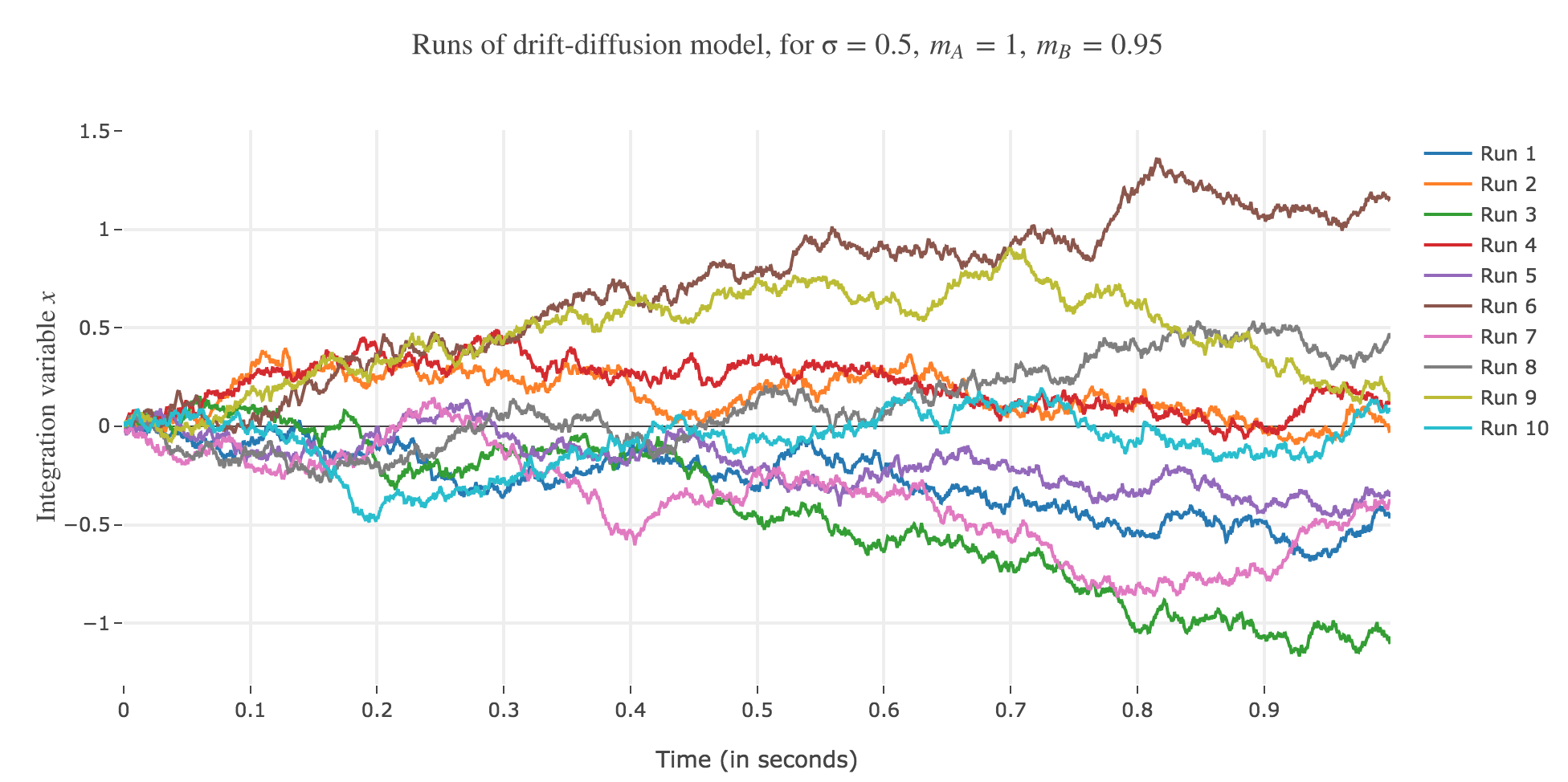Figure 3.1. - Ten runs of the drift-diffusion-model, with $m_A ≝ 1, m_B ≝ 0.95, \, σ ≝ 0.5, \, x(0) ≝ 0$

Now, after running the model $N$ times and storing the outcome and the time of threshold crossing (denoted by $t_i$) for each run: let us plot the distribution of reaction times for outcome $A$ and $B$, given that the reaction time is given by:

$RT_i = 100 + t_i$
• For $N ≝ 1000$: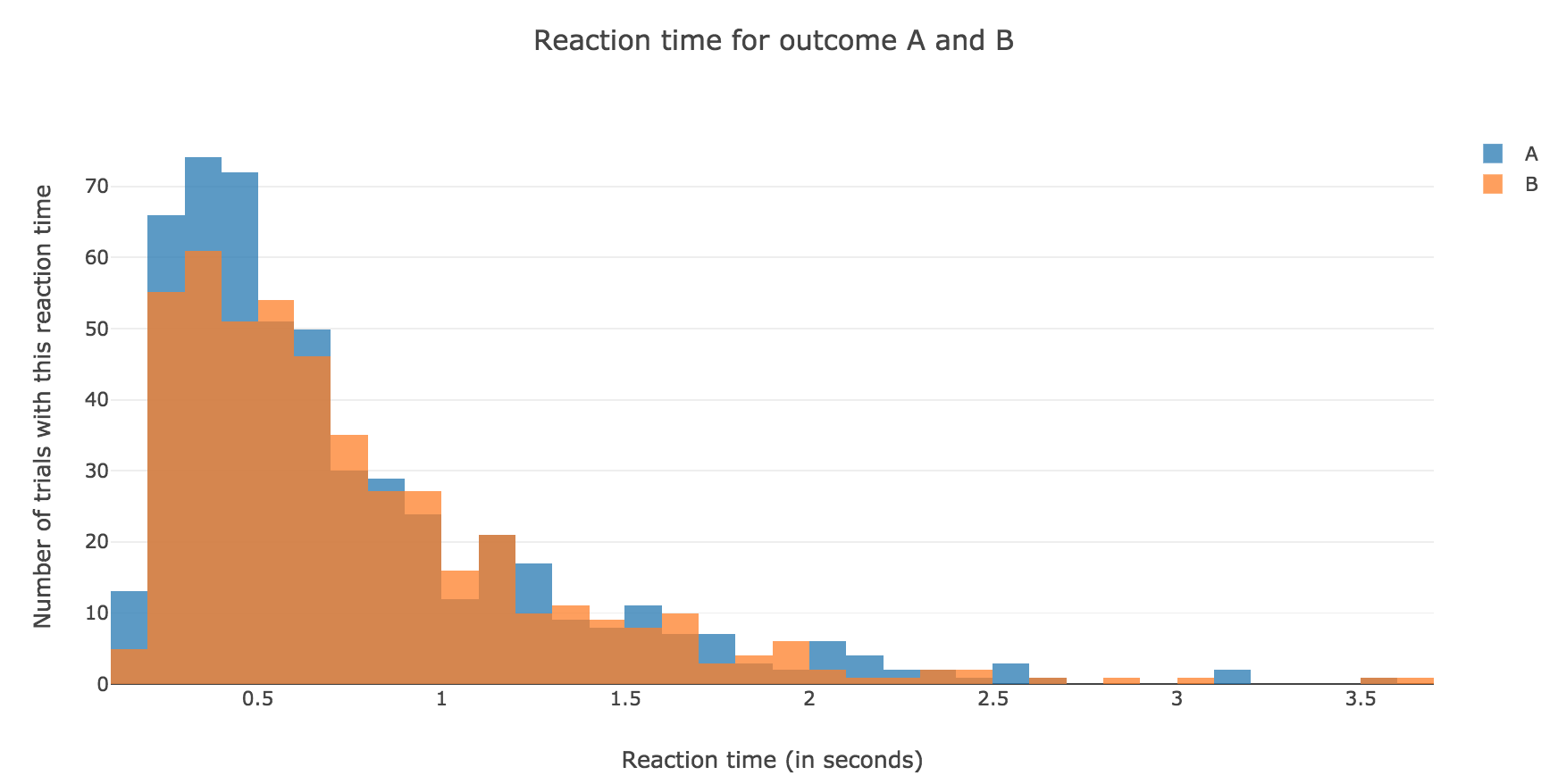Figure 1.1. - Distribution of reaction times for outcome $A$ and $B$ after running the model $N = 1000$ times
• For $N ≝ 10000$: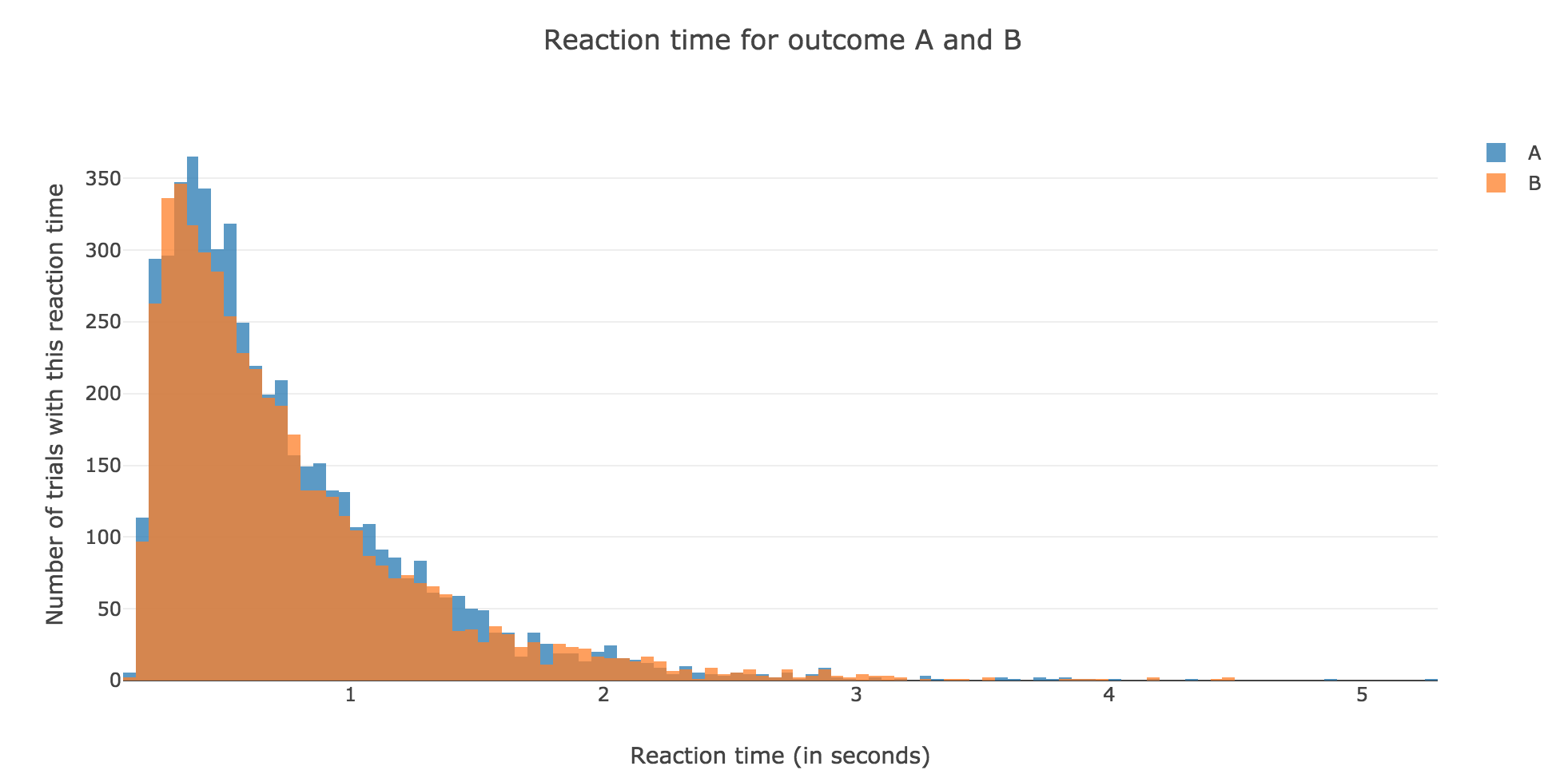Figure 1.2. - Distribution of reaction times for outcome $A$ and $B$ after running the model $N = 10000$ times

## 2. Probability of outcome $A$

Let us define the evidence for outcome $A$ versus outcome $B$ as:

$m_E ≝ m_A - m_B$

The analytical formula of the probability of outcome $A$ is:

$p_A ≝ \frac{1}{1+ \exp(-β m_E)}$

where $β ≝ \frac{2 μ}{σ^2}$.

Let us compare it, for values of $m_E$ ranging from $-0.2$ to $0.2$, with the empirical probability we can get from our simulation, by running the model $N$ times (for $N$ sufficiently large) and computing:

$P(A) ≝ \frac{\text{Number of trials of outcome } A}{\text{Number of trials of outcome } A + \text{Number of trials of outcome } B}$

The problem is that we have to compute this empirical probability for several values of $m_E$ ranging from $-0.2$ to $0.2$! As a matter of fact: the previous naive algorithm, based on the Euler method, is far too slow…

But we can cope with that with a trick!

### Fast algorithm to compute the distribution of reaction times

From the discrete approximation of the drift-diffusion-model:

$x(t+∆t) = x(t) + m_E ∆t + σ η(t) \sqrt{∆t}$

So for all $n ≥ 1$:

$x(t+ n ∆t) = x(0) + n \cdot m_E ∆t + σ \sqrt{∆t} \sum\limits_{ i=1 }^n η_i(t)$

where the

$η_i(t) \sim 𝒩(\overbrace{0}^{ ≝ \, μ_i}, \overbrace{1}^{≝ \, σ_i^2})$

are independent and normally distributed random variables.

But it is well known that a sum of independent and normally distributed random variables is also a normally distributed variable, such that:

$\sum\limits_{ i=1 }^n η_i(t) \sim 𝒩\left(\sum\limits_{ i=1 }^n μ_i \, , \; \sum\limits_{ i=1 }^n σ_i^2\right)$

i.e.

$\sum\limits_{ i=1 }^n η_i(t) \sim 𝒩(0, n)$

But as it happens:

$\sqrt{n} \underbrace{η(t)}_{\sim 𝒩(0, 1)} \sim 𝒩(0, n)$

So for all $n ≥ 1$, we can set $x(t + n ∆t)$ to be:

$x(t + n ∆t) = x(0) + n \cdot m_E ∆t + \sqrt{n} \cdot σ \sqrt{∆t} η(t)$

where $η(t) \sim 𝒩(0, 1)$

So solving for $n$ in the threshold crossing conditions:

$x(t + n ∆t) = ± μ$

is tantamount to:

• Analysis: solving (for $ξ$, in $ℂ$) two quadratic equations for a given $η(t) ∈ ℝ$:

$\begin{cases} m_E ∆t \, ξ^2 + σ \sqrt{∆t} η(t) \, ξ + x(0) - μ = 0&&\text{(outcome A)}\\ m_E ∆t \, ξ^2 + σ \sqrt{∆t} η(t) \, ξ + x(0) + μ = 0&&\text{(outcome A)}\\ \end{cases}$
• Synthesis: keeping only the roots $ξ$ such that $ξ^2 ∈ ℝ_+$, and setting $n ≝ \lceil ξ^2 \rceil$

which gives us a very fast algorithm!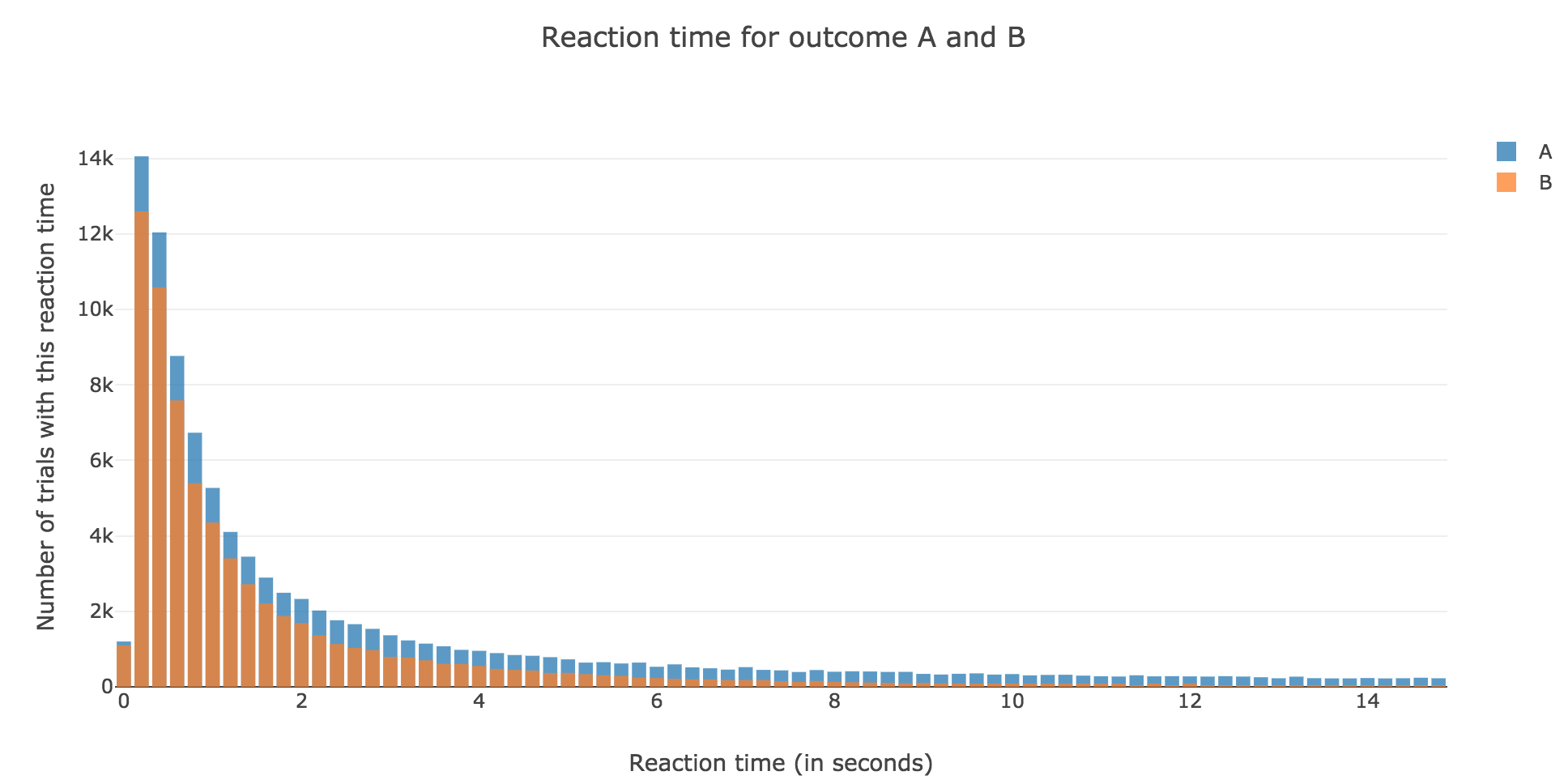Figure 2.1. - Distribution of reaction times for outcome $A$ and $B$ computed with the previous trick

Finally, we can compare the empirical probability of outcome $A$ with the analytical one: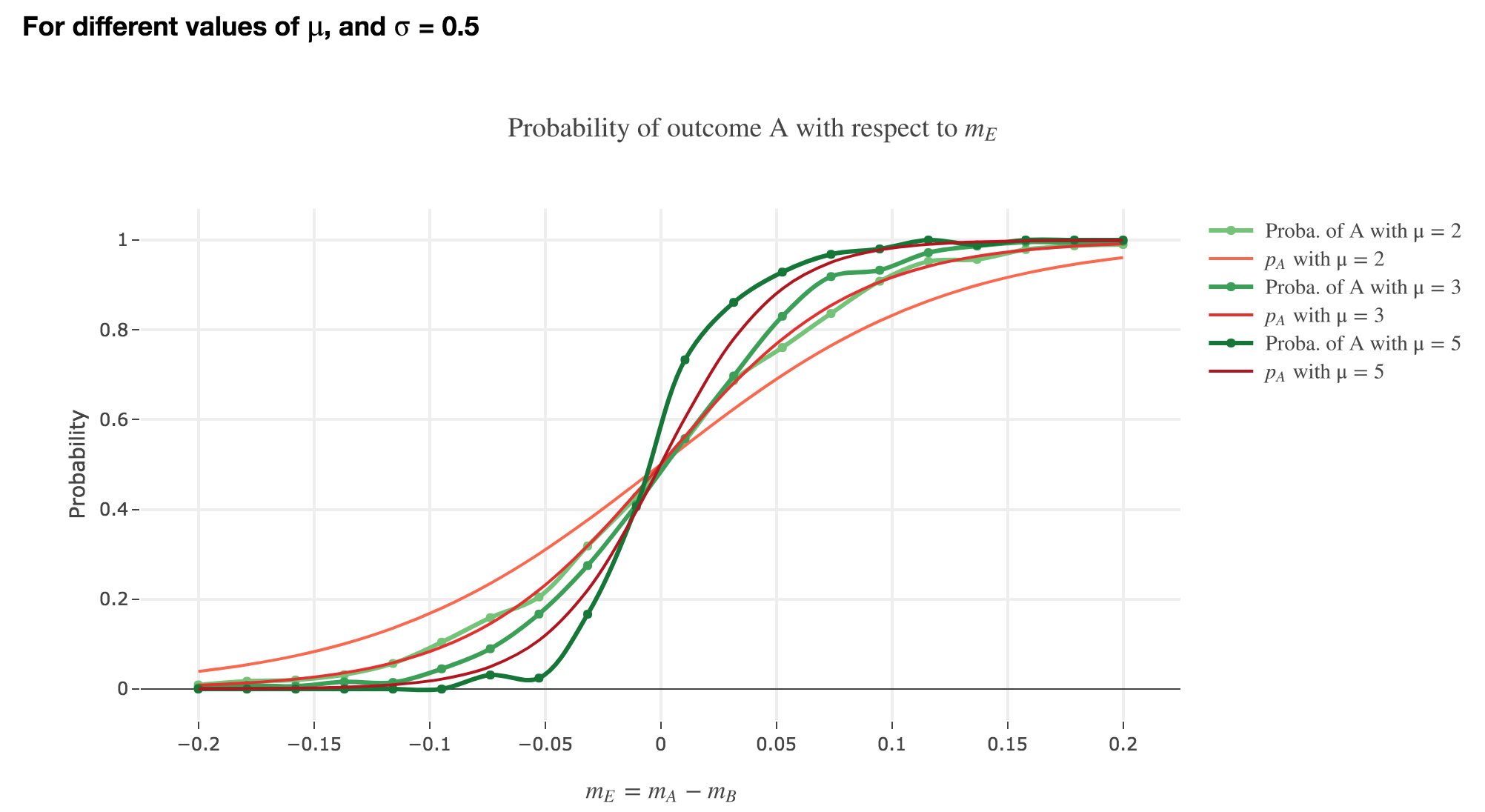Figure 2.2. - Comparison of the empirical probability of outcome $A$ with $p_A$ (as functions of $m_E$), for different values of $μ$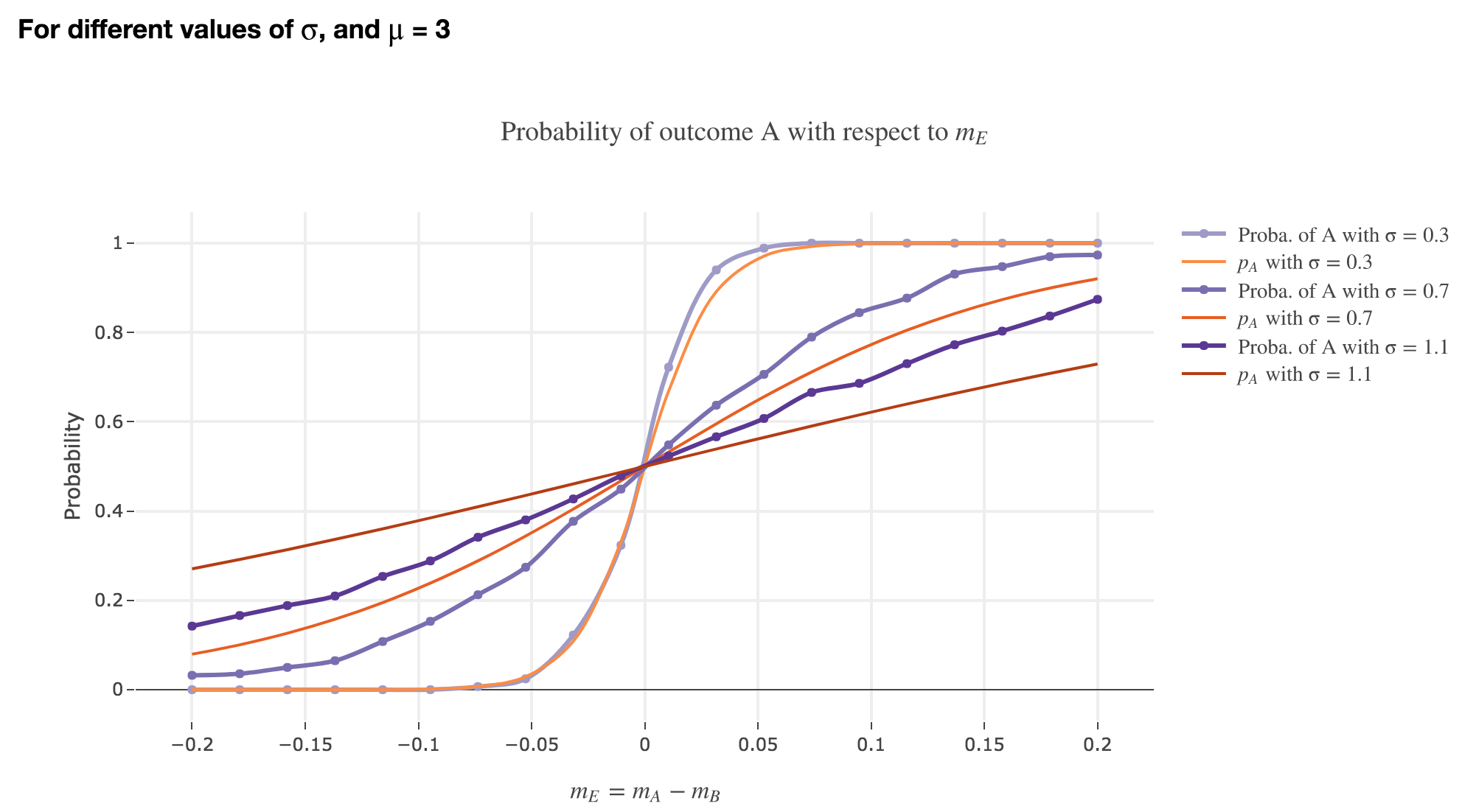Figure 2.3. - Comparison of the empirical probability of outcome $A$ with $p_A$ (as functions of $m_E$), for different values of $μ$

We see that the empirical probability of outcome $A$ matches more and more $p_A$ (when it comes to the shape of the curve):

• as $μ$ increases

• as $σ$ decreases (with an almost perfect match above for $σ = 0.3$)

Tags:

Updated: